# Chart Types in Xamarin Charts (SfChart)

24 Nov 202224 minutes to read

## Line Chart

To render a `Xamarin Line chart`, create an instance of `LineSeries` and add to the `Series` collection property of `SfChart`. You can use the following properties to customize the appearance.

``````<chart:SfChart>
...

<chart:LineSeries ItemsSource ="{Binding Data}" XBindingPath="Year"
YBindingPath="Value"/>

</chart:SfChart>``````
``````SfChart chart = new SfChart();
...

LineSeries lineSeries = new LineSeries()
{
ItemsSource = Data,
XBindingPath = "Year",
YBindingPath = "Value"
};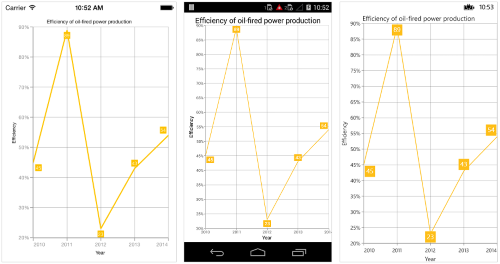NOTE

You can also explore our Xamarin Line Chart example to know how to represent time-dependent data, showing trends in data at equal intervals.

### Dashed Lines

`StrokeDashArray` property of the `LineSeries` is used to render line series with dashes.

``````<chart:LineSeries ItemsSource ="{Binding Data}" XBindingPath="Month" YBindingPath="Value">
<chart:LineSeries.StrokeDashArray>
<x:Array Type="{x:Type x:Double}">
<sys:Double>5</sys:Double>
<sys:Double>6</sys:Double>
</x:Array>
</chart:LineSeries.StrokeDashArray>
</chart:LineSeries>``````
``````LineSeries lineSeries = new LineSeries()
{
ItemsSource = Data,
XBindingPath = "Month",
YBindingPath = "Value"
};

lineSeries.StrokeDashArray = new double { 5, 6 };

## Fast Line Chart

`FastLineSeries` is a line chart, but it loads faster than `LineSeries`. You can use this when there are large number of points to be loaded in chart. To render a fast line chart, create an instance of `FastLineSeries` and add to the `Series` collection property of `SfChart`. You can use the following properties to customize the fast line segment appearance.

``````<chart:SfChart>
...

<chart:FastLineSeries ItemsSource ="{Binding Data}" XBindingPath="XValue" YBindingPath="YValue"/>

</chart:SfChart>``````
``````SfChart chart = new SfChart();
...

FastLineSeries fastLineSeries = new FastLineSeries()
{
ItemsSource = Data,
XBindingPath = "XValue",
YBindingPath = "YValue"
};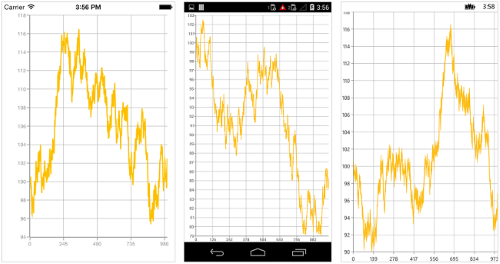### Dashed Lines

`StrokeDashArray` property of the `FastLineSeries` is used to render fast line series with dashes.

• C#
• ``````[C#]

FastLineSeries fastLineSeries = new FastLineSeries()
{
ItemsSource = Data,
XBindingPath = "Month",
YBindingPath = "Value"
};
fastLineSeries.StrokeDashArray = new double { 2, 3 };``````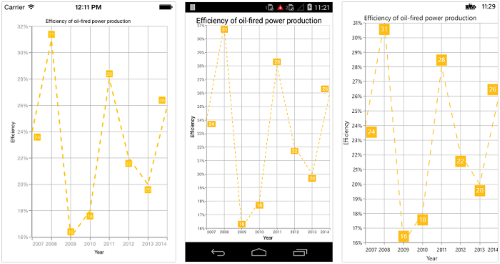### EnableAntiAliasing

Since `FastLineSeries` can be loaded with a large number of points, the rendering of series should be smooth. This requirement can be achieved by setting `EnableAntiAliasing` property of `FastLineSeries` as false.

## Stacked Line Chart

To render a stacked line chart, create an instance of `StackingLineSeries` and add to the `Series` collection property of `SfChart`. You can use the following properties to customize the stacked line appearance.

``````<chart:SfChart>
...

<chart:StackingLineSeries ItemsSource ="{Binding Data1}" XBindingPath="XValue" YBindingPath="YValue"/>

<chart:StackingLineSeries ItemsSource ="{Binding Data2}" XBindingPath="XValue" YBindingPath="YValue"/>

<chart:StackingLineSeries ItemsSource ="{Binding Data3}" XBindingPath="XValue" YBindingPath="YValue"/>

<chart:StackingLineSeries ItemsSource ="{Binding Data4}" XBindingPath="XValue" YBindingPath="YValue"/>

</chart:SfChart>``````
``````SfChart chart = new SfChart();
...

StackingLineSeries stackingLineSeries1 = new StackingLineSeries()
{
ItemsSource = Data1,
XBindingPath = "XValue",
YBindingPath = "YValue"
};

StackingLineSeries stackingLineSeries2 = new StackingLineSeries()
{
ItemsSource = Data2,
XBindingPath = "XValue",
YBindingPath = "YValue"
};

StackingLineSeries stackingLineSeries3 = new StackingLineSeries()
{
ItemsSource = Data3,
XBindingPath = "XValue",
YBindingPath = "YValue"
};

StackingLineSeries stackingLineSeries4 = new StackingLineSeries()
{
ItemsSource = Data4,
XBindingPath = "XValue",
YBindingPath = "YValue"
};

### Dashed Stacked Lines

`StrokeDashArray` property of the `StackingLineSeries` is used to render stacked line series with dashes.

• C#
• ``````[C#]

StackingLineSeries stackingLineSeries1 = new StackingLineSerie()
{
ItemsSource = Data1,
XBindingPath = "XValue",
YBindingPath = "YValue"
};
stackingLineSeries1.StrokeDashArray = new double { 13, 4 };

StackingLineSeries stackingLineSeries2 = new StackingLineSerie()
{
ItemsSource = Data1,
XBindingPath = "XValue",
YBindingPath = "YValue"
};
stackingLineSeries2.StrokeDashArray = new double { 13, 4 };

StackingLineSeries stackingLineSeries3 = new StackingLineSerie()
{
ItemsSource = Data1,
XBindingPath = "XValue",
YBindingPath = "YValue"
};
stackingLineSeries3.StrokeDashArray = new double { 13, 4 };

StackingLineSeries stackingLineSeries4 = new StackingLineSerie()
{
ItemsSource = Data1,
XBindingPath = "XValue",
YBindingPath = "YValue"
};
stackingLineSeries4.StrokeDashArray = new double { 13, 4 };``````

## 100% Stacked Line Chart

To render a 100% stacked line chart, create an instance of `StackingLine100Series` and add to the `Series` collection property of `SfChart`. You can use the following properties to customize the series appearance.

``````<chart:SfChart>
...

<chart:StackingLine100Series ItemsSource ="{Binding Data1}" XBindingPath="XValue" YBindingPath="YValue"/>

<chart:StackingLine100Series ItemsSource ="{Binding Data2}" XBindingPath="XValue" YBindingPath="YValue"/>

<chart:StackingLine100Series ItemsSource ="{Binding Data3}" XBindingPath="XValue" YBindingPath="YValue"/>

<chart:StackingLine100Series ItemsSource ="{Binding Data4}" XBindingPath="XValue" YBindingPath="YValue"/>

</chart:SfChart>``````
``````SfChart chart = new SfChart();
...

StackingLine100Series stackingLine100Series1 = new StackingLine100Series()
{
ItemsSource = Data1,
XBindingPath = "XValue",
YBindingPath = "YValue"
};

StackingLine100Series stackingLine100Series2 = new StackingLine100Series()
{
ItemsSource = Data2,
XBindingPath = "XValue",
YBindingPath = "YValue"
};

StackingLine100Series stackingLine100Series3 = new StackingLine100Series()
{
ItemsSource = Data3,
XBindingPath = "XValue",
YBindingPath = "YValue"
};

StackingLine100Series stackingLine100Series4 = new StackingLine100Series()
{
ItemsSource = Data4,
XBindingPath = "XValue",
YBindingPath = "YValue"
};

## Area Chart

To render an `Xamarin Area Chart`, create an instance of `AreaSeries` and add to the `Series` collection property of `SfChart`. You can use the following properties to customize the appearance.

``````<chart:SfChart>
...

<chart:AreaSeries ItemsSource ="{Binding Data}" XBindingPath="Year"
YBindingPath="Value"/>

</chart:SfChart>``````
``````SfChart chart = new SfChart();
...

AreaSeries areaSeries = new AreaSeries()
{
ItemsSource = Data,
XBindingPath = "Year",
YBindingPath = "Value"
};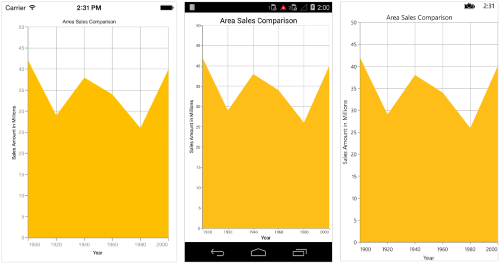NOTE

You can also explore our Xamarin Area Chart example to knows how to represent time-dependent data, showing trends in data at equal intervals.

## Spline Area Chart

To render a `Xamarin Spline Area chart`, create an instance of `SplineAreaSeries` and add to the `Series` collection property of `SfChart`. You can use the following properties to customize the spline area appearance.

``````<chart:SfChart>
...

<chart:SplineAreaSeries ItemsSource ="{Binding Data}" XBindingPath="Year"
YBindingPath="Value"/>

</chart:SfChart>``````
``````SfChart chart = new SfChart();
...

SplineAreaSeries splineAreaSeries = new SplineAreaSeries()
{
ItemsSource = Data,
XBindingPath = "Year",
YBindingPath = "Value"
};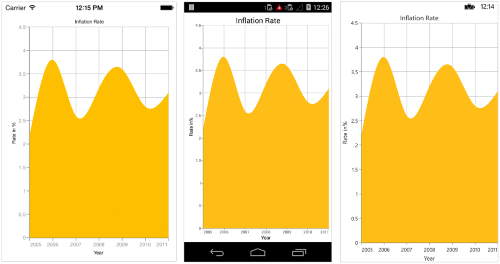NOTE

You can also explore our Xamarin Spline Area Chart example to knows how to represent time-dependent data and show trends in data at equal intervals.

### Spline Rendering Types

`SplineType` allows you to change the spline area curve in series.
The following types are used in `SplineAreaSeries` as

By default `SplineType` value is `Natural`.

The following code shows how to set the `SplineType` value as `Cardinal`

``````<chart:SfChart>
...

<chart:SplineAreaSeries ItemsSource ="{Binding Data}" XBindingPath="Month"
YBindingPath="Value" SplineType="Cardinal" />

</chart:SfChart>``````
``````SfChart chart = new SfChart();
...

SplineAreaSeries splineAreaSeries = new SplineAreaSeries()
{
ItemsSource = Data,
XBindingPath = "Month",
YBindingPath = "Value",
SplineType = SplineType.Cardinal
};

## Step Area Chart

To render a `Step Area chart`, create an instance of `StepAreaSeries` and add to the `Series` collection property of `SfChart`. You can use the following properties to customize the appearance.

``````<chart:SfChart>
...

<chart:StepAreaSeries ItemsSource ="{Binding Data}" XBindingPath="Year"
YBindingPath="Value"/>

</chart:SfChart>``````
``````SfChart chart = new SfChart();
...

StepAreaSeries stepAreaSeries = new StepAreaSeries()
{
ItemsSource = Data,
XBindingPath = "Year",
YBindingPath = "Value"
};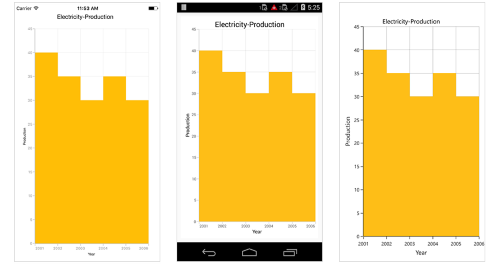## Range Area Chart

To render a `Xamarin Range Area chart`, create an instance of `RangeAreaSeries` and add to the `Series` collection property of `SfChart`.

Since the `RangeAreaSeries` requires two Y values for a point, your data should contain high and low values. High and low value specifies the maximum and minimum range of the point.

There are two ways you can provide data to range area chart,

1.You can use `ChartDataPoint's` three parameter constructor to pass `XValue`, `High` and `Low` values to `RangeAreaSeries`,

• C#
• ``````[C#]

SfChart chart = new SfChart();
...

ObservableCollection<ChartDataPoint> data = new ObservableCollection<ChartDataPoint>()
{
new ChartDataPoint("Jan/10", 30, 18),
new ChartDataPoint("Feb/10", 24, 12),
new ChartDataPoint("Mar/10", 29, 15),
new ChartDataPoint("Apr/10", 24, 10),
new ChartDataPoint("May/10", 30, 18),
new ChartDataPoint("Jun/10", 24, 10),
};

RangeAreaSeries rangeAreaSeries = new RangeAreaSeries()
{
ItemsSource = data
};

2.Or else you can use `High` and `Low` properties of `RangeAreaSeries` to map the high and low values from custom object to chart.

``````<chart:SfChart>
...
<chart:SfChart.Series>
<chart:RangeAreaSeries ItemsSource="{Binding RangeAreaData}" XBindingPath="Name" High="High" Low="Low"/>
</chart:SfChart.Series>
</chart:SfChart>``````
``````SfChart chart = new SfChart();
...

RangeAreaSeries rangeAreaSeries = new RangeAreaSeries()
{
XBindingPath = "Name",
High = "High",
Low = "Low"
};

You can use the following properties to customize the appearance.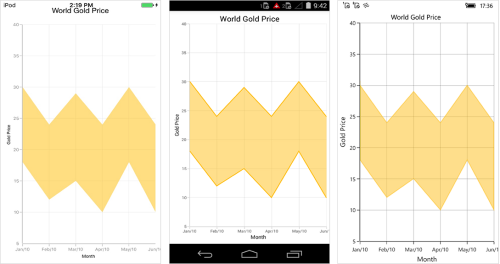NOTE

You can also explore our Xamarin Range Area Chart example to know how to show variations in the data values for a given time.

## Spline Range Area Chart

To render a `Xamarin Spline Range Area chart`, create an instance of the `SplineRangeAreaSeries`, and add that instance to the `Series` collection property of `SfChart`.

Since the `SplineRangeAreaSeries` requires two Y values for a point, data should contain high and low values. The high and low values specify the maximum and minimum ranges of a point.

The data can be provided to a spline range area chart by using the following two ways:

1.Using the `ChartDataPoint’s` three parameter constructor to pass `XValue`, `High`, and `Low` values to the `SplineRangeAreaSeries`.

• C#
• ``````[C#]

SfChart chart = new SfChart();
...

ObservableCollection<ChartDataPoint> data = new ObservableCollection<ChartDataPoint>()
{
new ChartDataPoint("Jan", 30, 18),
new ChartDataPoint("Feb", 24, 12),
new ChartDataPoint("Mar", 29, 15),
new ChartDataPoint("Apr", 24, 10),
new ChartDataPoint("May", 30, 18),
new ChartDataPoint("Jun", 24, 10),
};

SplineRangeAreaSeries splineRangeAreaSeries = new SplineRangeAreaSeries()
{
ItemsSource = data
};

2.Or else, using the `high` and `low` properties of `SplineRangeAreaSeries` to map the high and low values from custom object to chart.

``````<chart:SfChart>
...
<chart:SfChart.Series>
<chart:SplineRangeAreaSeries ItemsSource="{Binding SplineRangeAreaData}" XBindingPath="Name" High="High" Low="Low"/>
</chart:SfChart.Series>
</chart:SfChart>``````
``````SfChart chart = new SfChart();
...

SplineRangeAreaSeries splineRangeAreaSeries = new SplineRangeAreaSeries()
{
XBindingPath = "Name",
High = "High",
Low = "Low"
};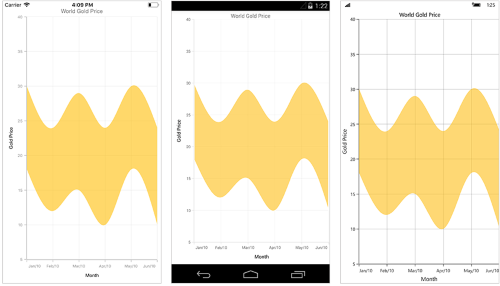NOTE

You can also explore our Xamarin Spline Range Area Chart example to knows how to display continuous data points as a set of splines that vary between high and low values.

### Spline Rendering Types

`SplineType` property allows you to change the spline range area curve in series.

The following types can be used for SplineRangeAreaSeries

• Natural
• Monotonic
• Cardinal
• Clamped

By default `SplineType` value is Natural.

The following code shows how to set the `SplineType` value as Cardinal.

``````<chart:SfChart>
…
<chart:SplineRangeAreaSeries ItemsSource="{Binding SplineRangeAreaData}" XBindingPath="Name" High="High" Low="Low" SplineType="Cardinal"/>

</chart:SfChart>``````
``````SplineRangeAreaSeries splineRangeAreaSeries = new SplineRangeAreaSeries
{
XBindingPath = "Name",
High = "High",
Low = "Low",
SplineType = SplineType.Cardinal
};

## Stacked Area Chart

To render a `Xamarin Stacked Area chart`, create an instance of `StackingAreaSeries` and add to the `Series` collection property of `SfChart`. You can use the following properties to customize the stacked area appearance.

``````<chart:SfChart>
...

<chart:StackingAreaSeries ItemsSource ="{Binding Data1}" XBindingPath="Year"
YBindingPath="Value"/>

<chart:StackingAreaSeries ItemsSource ="{Binding Data2}" XBindingPath="Year"
YBindingPath="Value"/>

<chart:StackingAreaSeries ItemsSource ="{Binding Data3}" XBindingPath="Year"
YBindingPath="Value"/>

</chart:SfChart>``````
``````SfChart chart = new SfChart();
...

StackingAreaSeries stackingAreaSeries1 = new StackingAreaSeries()
{
ItemsSource = Data1,
XBindingPath = "Year",
YBindingPath = "Value"
};

StackingAreaSeries stackingAreaSeries2 = new StackingAreaSeries()
{
ItemsSource = Data2,
XBindingPath = "Year",
YBindingPath = "Value"
};

StackingAreaSeries stackingAreaSeries3 = new StackingAreaSeries()
{
ItemsSource = Data3,
XBindingPath = "Year",
YBindingPath = "Value"
};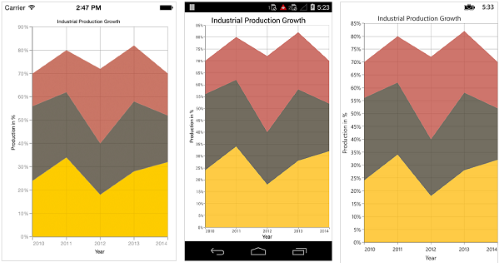NOTE

You can also explore our Xamarin Stacked Area Chart example to knows how to visualizes data with y-values stacked one over another in a series order.

## 100% Stacked Area Chart

To render a `Xamarin.Forms 100% Stacked Area chart`, create an instance of `StackingArea100Series` and add to the `Series` collection property of `SfChart`. You can use the following properties to customize the 100% stacked area appearance.

``````<chart:SfChart>
...

<chart:StackingArea100Series ItemsSource ="{Binding Data1}" XBindingPath="Year"
YBindingPath="Value"/>

<chart:StackingArea100Series ItemsSource ="{Binding Data2}" XBindingPath="Year"
YBindingPath="Value"/>

<chart:StackingArea100Series ItemsSource ="{Binding Data3}" XBindingPath="Year"
YBindingPath="Value"/>

</chart:SfChart>``````
``````SfChart chart = new SfChart();
...

StackingArea100Series stackingArea100Series1 = new StackingArea100Series()
{
ItemsSource = Data1,
XBindingPath = "Year",
YBindingPath = "Value"
};

StackingArea100Series stackingArea100Series2 = new StackingArea100Series()
{
ItemsSource = Data2,
XBindingPath = "Year",
YBindingPath = "Value"
};

StackingArea100Series stackingArea100Series3 = new StackingArea100Series()
{
ItemsSource = Data3,
XBindingPath = "Year",
YBindingPath = "Value"
};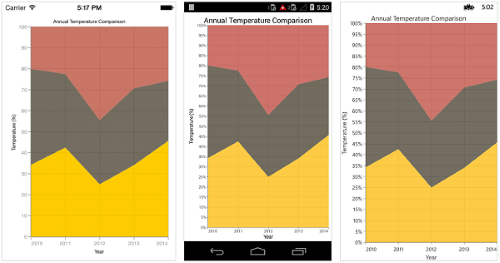NOTE

You can also explore our Xamarin.Forms Stacked Area 100% Chart example to knows how to displays multiple series of data as stacked areas, ensuring that the cumulative proportion of each stacked element always totals 100%.

## Column Chart

To render a `Xamarin Column Chart`, create an instance of `ColumnSeries` and add to the `Series` collection property of `SfChart`. You can use the following properties to customize the appearance.

``````<chart:SfChart>
...

<chart:ColumnSeries ItemsSource ="{Binding Data}" XBindingPath="Country"
YBindingPath="Value"/>

</chart:SfChart>``````
``````SfChart chart = new SfChart();
...

ColumnSeries columnSeries = new ColumnSeries()
{
ItemsSource = Data,
XBindingPath = "Country",
YBindingPath = "Value"
};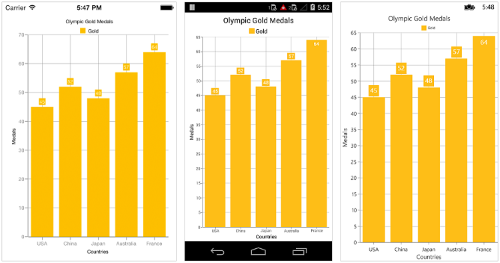NOTE

You can also explore our Xamarin Column Chart example how to create a column chart with data labels and customize its appearance with built-in color palette.

### Overlapped placement

By default, all the column series which has the same x and y axes are placed side by side in a chart. If you want place the series one over the other (overlapped), set the `SideBySideSeriesPlacement` property of `SfChart` to false and configure the `Width` property to differentiate the series. The following code snippet and screenshot illustrate the overlapped placement of column series.

``````<chart:SfChart x:Name="Chart" SideBySideSeriesPlacement="False" >
. . .
<chart:SfChart.Series>
<chart:ColumnSeries ItemsSource="{Binding Data1}" Label="2014" XBindingPath="Month" YBindingPath="Year2014">

</chart:ColumnSeries>
<chart:ColumnSeries  Width="0.5" ItemsSource="{Binding Data2}" Label="2015" XBindingPath="Month" YBindingPath="Year2015" >

</chart:ColumnSeries>
</chart:SfChart.Series>
. . .
</chart:SfChart>``````
``````SfChart chart = new SfChart()
{
SideBySideSeriesPlacement = false
};
chart.PrimaryAxis = new CategoryAxis();
chart.SecondaryAxis = new NumericalAxis();
ColumnSeries series1 = new ColumnSeries()
{
ItemsSource = view.Data1,
XBindingPath = "Month",
YBindingPath = "Year2014"
};
ColumnSeries series2 = new ColumnSeries()
{
ItemsSource = view.Data2,
XBindingPath = "Month",
YBindingPath = "Year2015",
Width="0.5"
};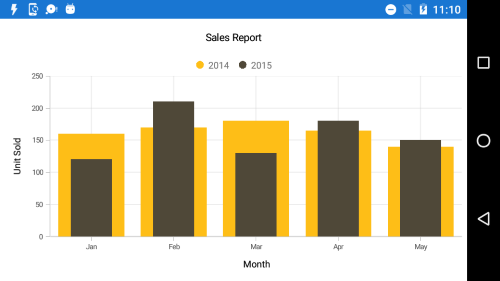## Histogram Chart

To render a `Xamarin Histogram chart`, create an instance of `HistogramSeries`, and add it to the series collection of `SfChart`.

Histogram chart provides a visual display of large amount of data that are difficult to understand in a tabular or data grid form.

You can customize intervals using the `Interval` property and collapse the normal distribution curve using the `ShowNormalDistributionCurve` property. You can use the following properties to customize the appearance.

``````<chart:SfChart>
...

<chart:HistogramSeries ItemsSource ="{Binding Data}" XBindingPath="XValue"
YBindingPath="YValue" Interval="20"/>

</chart:SfChart>``````
``````SfChart chart = new SfChart();
...

HistogramSeries histogramSeries = new HistogramSeries()
{
ItemsSource = Data,
XBindingPath = "XValue",
YBindingPath = "YValue",
Interval = 20
};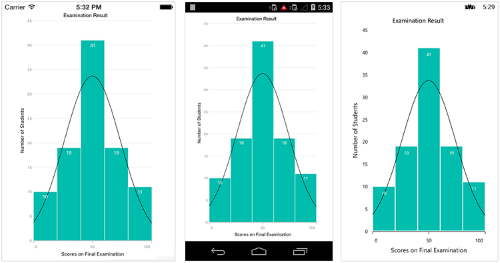NOTE

You can also explore our Xamarin Histogram Chart example to know how to represent time-dependent data, showing trends in data at equal intervals.

## Range Column Chart

To render a `Xamarin Range Column chart`, create an instance of `RangeColumnSeries` and add to the `Series` collection property of `SfChart`.

Since the `RangeColumnSeries` requires two Y values for a point, your data should contain high and low values. High and low value specifies the maximum and minimum range of the point.

There are two ways you can provide data to RangeColumn chart,

1.You can use `ChartDataPoint's` three parameter constructor to pass `XValue`, `High` and `Low` values to `RangeColumnSeries`,

• C#
• ``````[C#]

SfChart chart = new SfChart();
...

ObservableCollection<ChartDataPoint> data = new ObservableCollection<ChartDataPoint>()
{
new ChartDataPoint("Jan", 7.6, 1.8),
new ChartDataPoint("Feb", 10, 3),
new ChartDataPoint("Mar", 7.5, 1.7),
new ChartDataPoint("Apr", 7.8, 4.5),
new ChartDataPoint("May", 11.4, 5),
new ChartDataPoint("Jun", 10.1, 4.2),
};

RangeColumnSeries rangeColumnSeries = new RangeColumnSeries()
{
ItemsSource = data
};

2.Or else you can use `High` and `Low` properties of `RangeColumnSeries` to map the high and low values from custom object to chart.

``````<chart:SfChart>
...

<chart:RangeColumnSeries ItemsSource ="{Binding Data}"
XBindingPath="Month"
High="Value1"
Low="Value2"/>

</chart:SfChart>``````
``````SfChart chart = new SfChart();
...

RangeColumnSeries rangeColumnSeries = new RangeColumnSeries()
{
ItemsSource = Data,
XBindingPath = "Month",
High = "Value1",
Low = "Value2"
};

Following properties are used to customize the range column segment appearance,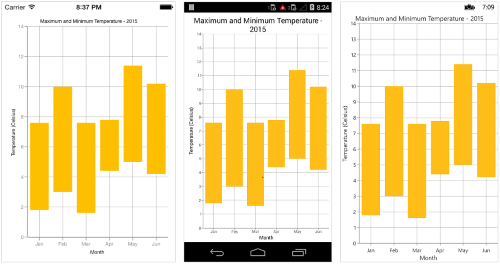NOTE

You can also explore our Xamarin Range Column Chart example to knows how to display a range of data by plotting two y-values per data point.

## Stacked Column Chart

To render a `Stacked Column chart`, create an instance of `StackingColumnSeries` and add to the `Series` collection property of `SfChart`. You can use the following properties to customize the stacked column segment appearance.

``````<chart:SfChart>
...

<chart:StackingColumnSeries ItemsSource ="{Binding Data1}" XBindingPath="Month"
YBindingPath="Value"/>

<chart:StackingColumnSeries ItemsSource ="{Binding Data2}" XBindingPath="Month"
YBindingPath="Value"/>

<chart:StackingColumnSeries ItemsSource ="{Binding Data3}" XBindingPath="Month"
YBindingPath="Value"/>

</chart:SfChart>``````
``````SfChart chart = new SfChart();
...

StackingColumnSeries stackingColumnSeries1 = new StackingColumnSeries()
{
ItemsSource = Data1,
XBindingPath = "Month",
YBindingPath = "Value"
};

StackingColumnSeries stackingColumnSeries2 = new StackingColumnSeries()
{
ItemsSource = Data2,
XBindingPath = "Month",
YBindingPath = "Value"
};

StackingColumnSeries stackingColumnSeries3 = new StackingColumnSeries()
{
ItemsSource = Data3,
XBindingPath = "Month",
YBindingPath = "Value"
};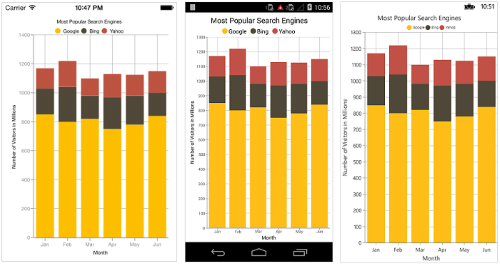## 100% Stacked Column Chart

To render a `100% Stacked Column chart`, create an instance of `StackingColumn100Series` and add to the `Series` collection property of `SfChart`. You can use the following properties to customize the series appearance.

``````<chart:SfChart>
...

<chart:StackingColumn100Series ItemsSource ="{Binding Data1}" XBindingPath="Year"
YBindingPath="Value"/>

<chart:StackingColumn100Series ItemsSource ="{Binding Data2}" XBindingPath="Year"
YBindingPath="Value"/>

<chart:StackingColumn100Series ItemsSource ="{Binding Data3}" XBindingPath="Year"
YBindingPath="Value"/>

</chart:SfChart>``````
``````SfChart chart = new SfChart();
...

StackingColumn100Series stackingColumn100Series1 = new StackingColumn100Series()
{
ItemsSource = Data1,
XBindingPath = "Year",
YBindingPath = "Value"
};

StackingColumn100Series stackingColumn100Series2 = new StackingColumn100Series()
{
ItemsSource = Data2,
XBindingPath = "Year",
YBindingPath = "Value"
};

StackingColumn100Series stackingColumn100Series3 = new StackingColumn100Series()
{
ItemsSource = Data3,
XBindingPath = "Year",
YBindingPath = "Value"
};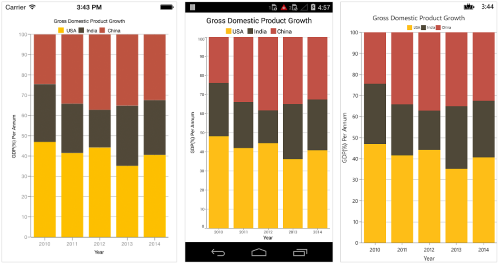## Bar Chart

To render a `Xamarin Bar Chart`, create an instance of `BarSeries` and add to the `Series` collection property of `SfChart`. You can use the following properties to customize the bar segment appearance.

``````<chart:SfChart>
...

<chart:BarSeries ItemsSource ="{Binding Data}" XBindingPath="Year"
YBindingPath="Value"/>

</chart:SfChart>``````
``````SfChart chart = new SfChart();
...

BarSeries barSeries = new BarSeries ()
{
ItemsSource = Data,
XBindingPath = "Year",
YBindingPath = "Value"
};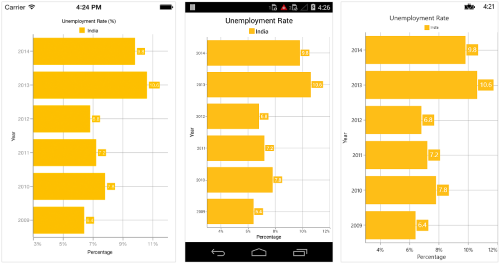NOTE

You can also explore our Xamarin Bar Chart example to compare values across categories by using horizontal bars.

## Stacked Bar Chart

To render a `Stacked Bar chart`, create an instance of `StackingBarSeries` and add to the `Series` collection property of `SfChart`. You can use the following properties to customize the stacked bar segment appearance.

``````<chart:SfChart>
...

<chart:StackingBarSeries ItemsSource ="{Binding Data1}" XBindingPath="Month"
YBindingPath="Value"/>

<chart:StackingBarSeries ItemsSource ="{Binding Data2}" XBindingPath="Month"
YBindingPath="Value"/>

<chart:StackingBarSeries ItemsSource ="{Binding Data3}" XBindingPath="Month"
YBindingPath="Value"/>

</chart:SfChart>``````
``````SfChart chart = new SfChart();
...

StackingBarSeries stackingBarSeries1 = new StackingBarSeries()
{
ItemsSource = Data1,
XBindingPath = "Month",
YBindingPath = "Value"
};

StackingBarSeries stackingBarSeries2 = new StackingBarSeries()
{
ItemsSource = Data2,
XBindingPath = "Month",
YBindingPath = "Value"
};

StackingBarSeries stackingBarSeries3 = new StackingBarSeries()
{
ItemsSource = Data3,
XBindingPath = "Month",
YBindingPath = "Value"
};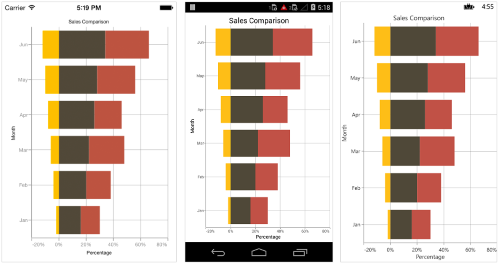## 100% Stacked Bar Chart

To render a `Xamarin 100% Stacked Bar chart`, create an instance of `StackingBar100Series` and add to the `Series` collection property of `SfChart`. You can use the following properties to customize the series appearance.

``````<chart:SfChart>
...

<chart:StackingBar100Series ItemsSource ="{Binding Data1}" XBindingPath="Year"
YBindingPath="Value"/>

<chart:StackingBar100Series ItemsSource ="{Binding Data2}" XBindingPath="Year"
YBindingPath="Value"/>

<chart:StackingBar100Series ItemsSource ="{Binding Data3}" XBindingPath="Year"
YBindingPath="Value"/>

</chart:SfChart>``````
``````SfChart chart = new SfChart();
...

StackingBar100Series stackingBar100Series1 = new StackingBar100Series()
{
ItemsSource = Data1,
XBindingPath = "Year",
YBindingPath = "Value"
};

StackingBar100Series stackingBar100Series2 = new StackingBar100Series()
{
ItemsSource = Data2,
XBindingPath = "Year",
YBindingPath = "Value"
};

StackingBar100Series stackingBar100Series3 = new StackingBar100Series()
{
ItemsSource = Data3,
XBindingPath = "Year",
YBindingPath = "Value"
};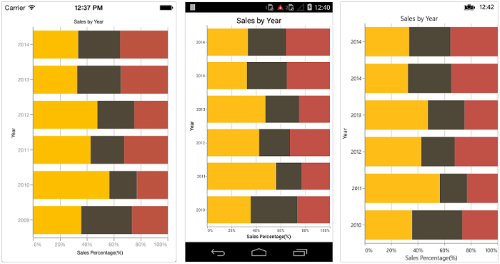NOTE

You can also explore our Xamarin Stacked Bar 100% Chart example to knows how to displays multiple series of data as stacked bars.

## Spline Chart

To render a `Xamarin Spline chart`, create an instance of `SplineSeries` and add to the `Series` collection property of `SfChart`. You can use the following properties to customize the spline segment appearance.

``````<chart:SfChart>
...

<chart:SplineSeries ItemsSource ="{Binding Data}" XBindingPath="Month"
YBindingPath="Value"/>

</chart:SfChart>``````
``````SfChart chart = new SfChart();
...

SplineSeries splineSeries = new SplineSeries()
{
ItemsSource = Data,
XBindingPath = "Month",
YBindingPath = "Value"
};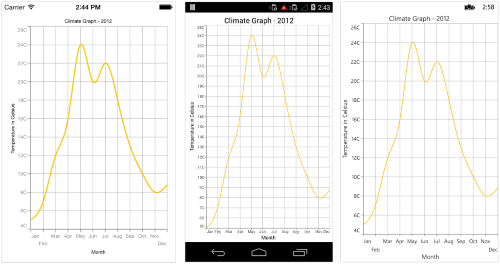NOTE

You can also explore our Xamarin Spline Chart example to knows how to connecting the data points with straight lines.

### Dashed Lines

`StrokeDashArray` property of the `SplineSeries` is used to render spline series with dashes.

``````<chart:SplineSeries ItemsSource ="{Binding Data}" XBindingPath="Month" YBindingPath="Value">
<chart:SplineSeries.StrokeDashArray>
<x:Array Type="{x:Type x:Double}">
<sys:Double>5</sys:Double>
<sys:Double>6</sys:Double>
</x:Array>
</chart:SplineSeries.StrokeDashArray>
</chart:SplineSeries>``````
``````SplineSeries splineSeries = new SplineSeries()
{
ItemsSource = Data,
XBindingPath = "Month",
YBindingPath = "Value"
};

splineSeries.StrokeDashArray = new double { 5, 6 };

### Spline Rendering Types

`SplineType` allows you to change the spline curve in series.
The following types are used in `SplineSeries` as

• Natural
• Monotonic
• Cardinal
• Clamped

By default `SplineType` value is `Natural`.

The following code shows how to set the `SplineType` value as `Cardinal`

``````<chart:SfChart>
...

<chart:SplineSeries ItemsSource ="{Binding Data}" XBindingPath="Month"
YBindingPath="Value" SplineType="Cardinal" />

</chart:SfChart>``````
``````SfChart chart = new SfChart();
...

SplineSeries splineSeries = new SplineSeries()
{
ItemsSource = Data,
XBindingPath = "Month",
YBindingPath = "Value",
SplineType = SplineType.Cardinal
};

`Cardinal`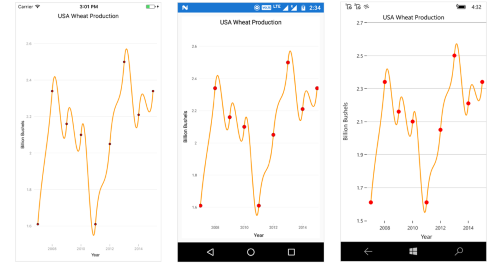`Monotonic`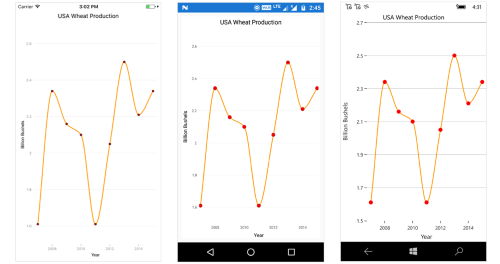`Clamped`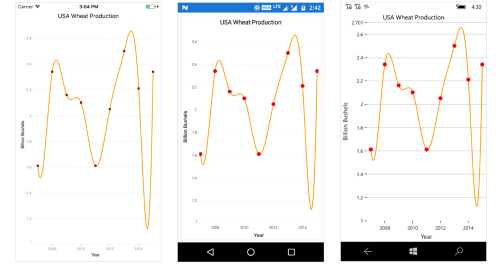## Step Line Chart

To render a `Step Line chart`, create an instance of `StepLineSeries` and add to the `Series` collection property of
`SfChart`. You can use the following properties to customize the spline segment appearance.

``````<chart:SfChart.Series>

<chart:StepLineSeries ItemsSource="{Binding Data}" XBindingPath="Month"
YBindingPath="Value" />

</chart:SfChart.Series>``````
``````SfChart chart = new SfChart();
...

StepLineSeries stepLine = new StepLineSeries()
{
ItemsSource = Data,
XBindingPath = "Month",
YBindingPath = "Value"
};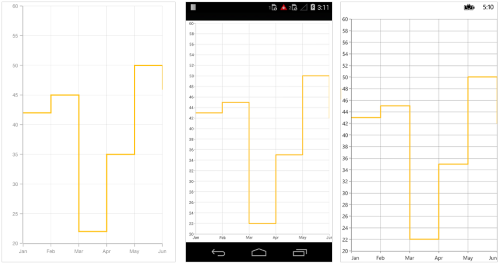## Bubble Chart

To render a `Xamarin Bubble Chart`, create an instance of `BubbleSeries` and add to the `Series` collection property of `SfChart`.

Bubble chart requires 3 fields (X, Y and Size) to plot a point. Here `Size` is used to specify the size of each bubble segment.

There are two ways you can provide data to bubble chart,

1.You can use ChartDataPoint’s three parameter constructor to pass `XValue`, `YValue` and `Size` values to `BubbleSeries`,

• C#
• ``````[C#]

SfChart chart = new SfChart();
...

ObservableCollection<ChartDataPoint> data = new ObservableCollection<ChartDataPoint>()
{
new ChartDataPoint(64, 14.4, 20),
new ChartDataPoint(71, 2, 15),
new ChartDataPoint(74, 7, 30),
new ChartDataPoint(80, 4, 22),
new ChartDataPoint(82, 10.3, 28),
new ChartDataPoint(94, 1, 8),
new ChartDataPoint(96, 6, 18),
new ChartDataPoint(98, 12.3, 28),
};

BubbleSeries bubbleSeries = new BubbleSeries()
{
ItemsSource = data
};

2.Or else you can use `YBindingPath` and `Size` properties of `BubbleSeries` to map the Y value and size from custom object to chart.

``````<chart:SfChart>
...

<chart:BubbleSeries ItemsSource ="{Binding Data}"
XBindingPath="XValue"
YBindingPath="YValue"
Size="Size"/>

</chart:SfChart>``````
``````SfChart chart = new SfChart();
...

BubbleSeries bubbleSeries = new BubbleSeries()
{
ItemsSource = Data,
XBindingPath = "XValue",
YBindingPath = "YValue",
Size = "Size”
};

Following properties are used to customize the bubble segment appearance.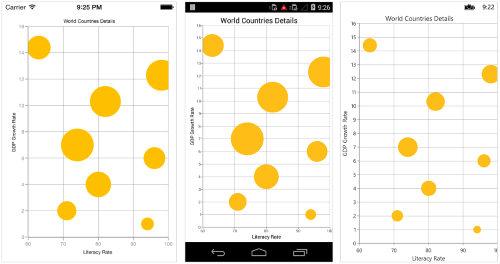### Show zero size bubbles

The zero size bubble segments can be enabled or disabled by using the `ShowZeroSizeBubbles` property. The default value of `ShowZeroSizeBubbles` property value is true.

``````<chart:SfChart.Series>
...

<chart:BubbleSeries ShowZeroSizeBubbles = "True"/>

</chart:SfChart>``````
``````SfChart chart = new SfChart();
...

BubbleSeries bubbleSeries = new BubbleSeries()
{
ShowZeroSizeBubbles = true;
};The following code example and screenshots describes when `ShowZeroSizeBubbles` value is false.

``````<chart:SfChart.Series>
...

<chart:BubbleSeries ShowZeroSizeBubbles = "False"/>

</chart:SfChart>``````
``````SfChart chart = new SfChart();
...

BubbleSeries bubbleSeries = new BubbleSeries()
{
ShowZeroSizeBubbles = false;
};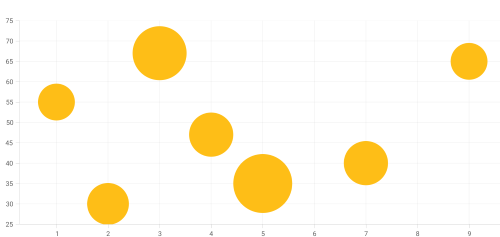NOTE

You can also explore our Xamarin Bubble Chart example to knows how to render and configure the bubble type charts

## Scatter Chart

To render a `Xamarin Scatter chart`, create an instance of `ScatterSeries` and add to the `Series` collection property of `SfChart`. You can use the following properties to customize the scatter segment appearance.

``````<chart:SfChart>
...

<chart:ScatterSeries ScatterHeight="15"
ScatterWidth="15"
ShapeType="Ellipse"
ItemsSource ="{Binding Data}"
XBindingPath="Year"
YBindingPath="Value"/>

</chart:SfChart>``````
``````SfChart chart = new SfChart();
...

ScatterSeries scatterSeries = new ScatterSeries ()
{
ItemsSource = Data,
ScatterHeight = 15,
ScatterWidth = 15,
ShapeType = ChartScatterShapeType.Ellipse,
XBindingPath = "Year",
YBindingPath = "Value"
};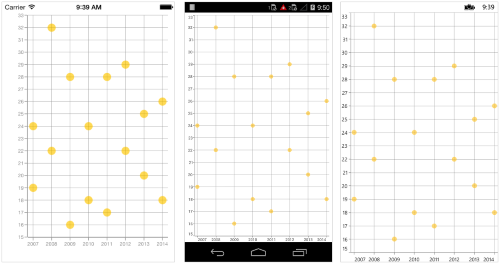NOTE

You can also explore our Xamarin Scatter Chart example to knows how to represent time-dependent data, showing trends in data at equal intervals.

## Fast Scatter Chart

The `FastScatterSeries` is a special kind of scatter series that renders a collection with a huge number of data points. You can use the following properties to customize the appearance of a fast scatter point.

``````<chart:SfChart>
...

<chart:FastScatterSeries ScatterHeight="15"
ScatterWidth="15"
ShapeType="Ellipse"
ItemsSource ="{Binding Data}"
XBindingPath="Year"
YBindingPath="Value"/>

</chart:SfChart>``````
``````SfChart chart = new SfChart();
...

FastScatterSeries fastScatterSeries = new FastScatterSeries ()
{
ItemsSource = Data,
ScatterHeight = 15,
ScatterWidth = 15,
ShapeType = ChartScatterShapeType.Ellipse,
XBindingPath = "Year",
YBindingPath = "Value"
};## OHLC Chart

To render an `Xamarin OHLC chart`, create an instance of `HiLoOpenCloseSeries` and add to the `Series` collection property of `SfChart`.

OHLC chart requires five values (X, Open, High, Low and Close) to plot a point.

There are two ways you can provide data to an OHLC chart,

1.You can use `ChartDataPoint's` five parameter constructor to pass `XValue`, `Open`, `High`, `Low`, `Close` , `Volume` values to `HiLoOpenCloseSeries` and `technical indicators`.

• C#
• ``````[C#]

SfChart chart = new SfChart();
...

ObservableCollection<ChartDataPoint> data = new ObservableCollection<ChartDataPoint>()
{
new ChartDataPoint("2010", 873.8, 878.85, 855.5, 860.5),
new ChartDataPoint("2011", 861, 868.4, 835.2, 843.45),
new ChartDataPoint("2012", 846.15, 853, 838.5, 847.5),
new ChartDataPoint("2013", 846, 860.75, 841, 855),
new ChartDataPoint("2014", 841, 845, 827.85, 838.65)
};

HiLoOpenCloseSeries hiLoOpenCloseSeries = new HiLoOpenCloseSeries()
{
ItemsSource = data
};

2.Or else you can use `Open`,`High`,`Low` and `Close` properties of `HiLoOpenCloseSeries` to map Open, High, Low and Close values from custom object to chart.

``````<chart:SfChart>
...

<chart:HiLoOpenCloseSeries ItemsSource ="{Binding Data}" XBindingPath="Year"
High="Value1" Low="Value2"
Open="Value3" Close="Value4"/>

</chart:SfChart>``````
``````SfChart chart = new SfChart();
...

HiLoOpenCloseSeries hiLoOpenCloseSeries = new HiLoOpenCloseSeries()
{
ItemsSource = Data,
XBindingPath = "Year",
Open = "Value1",
High = "Value2",
Low = "Value3",
Close = "Value4"
};

You can use the following properties to customize the `HiLoOpenCloseSeries` segment appearance.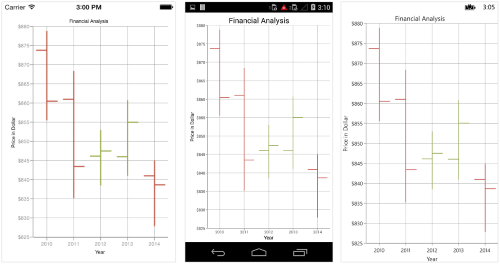NOTE

You can also explore our Xamarin OHLC Chart example to know how to represent time-dependent data, showing trends in data at equal intervals.

### Bull and Bear Color

In OHLC chart, `BullFillColor` property is used to specify a fill color for the segments that indicates an increase in stock price in the measured time interval and `BearFillColor` property is used to specify a fill color for the segments that indicates a decrease in stock price in the measured time interval.

``````<chart:HiLoOpenCloseSeries BearFillColor="Blue"
BullFillColor="Purple"
ItemsSource ="{Binding Data}"
XBindingPath="Year"
High="Value1"
Low="Value2"
Open="Value3"
Close="Value4"/>``````
``````HiLoOpenCloseSeries hiLoOpenCloseSeries = new HiLoOpenCloseSeries()
{
ItemsSource = Data,
XBindingPath = "Year",
Open = "Value1",
High = "Value2",
Low = "Value3",
Close = "Value4",
BearFillColor = Color.Blue,
BullFillColor = Color.Purple
};``````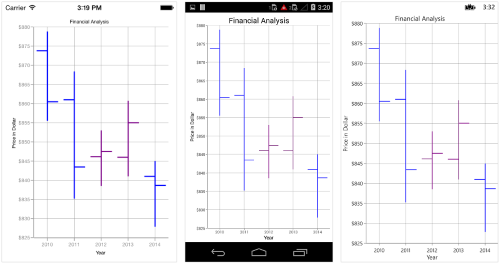## Candle Chart

To render a `Xamarin Candle Chart`, create an instance of `CandleSeries` and add to the `Series` collection property of `SfChart`.

Candle chart requires five values (X, Open, High, Low and Close) to plot a point.

There are two ways you can provide data to an candle chart,

1.You can use ChartDataPoint’s five parameter constructor to pass `XValue`, `Open`, `High`, `Low` and close values to `CandleSeries`,

• C#
• ``````[C#]

SfChart chart = new SfChart();
...

ObservableCollection<ChartDataPoint> data = new ObservableCollection<ChartDataPoint>()
{
new ChartDataPoint("2010", 873.8, 878.85, 855.5, 860.5),
new ChartDataPoint("2011", 861, 868.4, 835.2, 843.45),
new ChartDataPoint("2012", 846.15, 853, 838.5, 847.5),
new ChartDataPoint("2013", 846, 860.75, 841, 855),
new ChartDataPoint("2014", 841, 845, 827.85, 838.65)
};

CandleSeries candleSeries = new CandleSeries ()
{
ItemsSource = data
};

2.Or else you can use `Open`,`High`,`Low` and `Close` property of `CandleSeries` to map Open, High, Low and Close values from custom object to chart.

``````<chart:SfChart>
...

<chart:CandleSeries ItemsSource ="{Binding Data}"
XBindingPath="Year"
High="Value1"
Low="Value2"
Open="Value3"
Close="Value4"/>

</chart:SfChart>``````
``````SfChart chart = new SfChart();
...

CandleSeries candleSeries = new CandleSeries()
{
ItemsSource = Data,
XBindingPath = "Year",
Open = "Value1",
High = "Value2",
Low = "Value3",
Close = "Value4"
};

You can use the following properties to customize the candle segment appearance.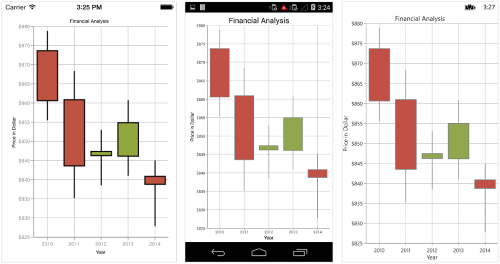NOTE

You can also explore our Xamarin Candle Chart example to knows how to represent time-dependent data, showing trends in data at equal intervals.

### Bull and Bear Color

In Candle chart, `BullFillColor` property is used to specify a fill color for the segments that indicates an increase in stock price in the measured time interval and `BearFillColor` property is used to specify a fill color for the segments that indicates a decrease in stock price in the measured time interval.

``````<chart:CandleSeries BearFillColor="Blue"
BullFillColor="Purple"
ItemsSource ="{Binding Data}"
XBindingPath="Year"
High="Value1"
Low="Value2"
Open="Value3"
Close="Value4" />``````
``````CandleSeries candleSeries = new CandleSeries()
{
ItemsSource = Data,
XBindingPath = "Year",
Open = "Value1",
High = "Value2",
Low = "Value3",
Close = "Value4",
BearFillColor = Color.Blue,
BullFillColor = Color.Purple
};``````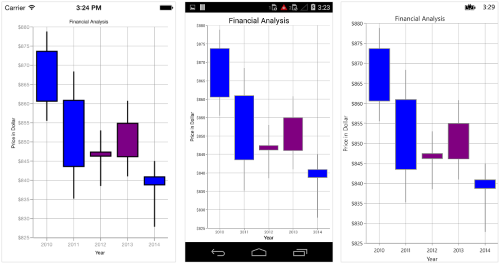### EnableSolidCandles

In Candle Series, `EnableSolidCandles` property is used to specify whether the candle segment should be filled or hollow. The default value of this property is false.

``````<chart:SfChart>
...
<chart:SfChart.Series>

<chart:CandleSeries ItemsSource="{Binding FinancialData}" EnableSolidCandles="True"/>

</chart:SfChart.Series>

</chart:SfChart>``````
``````SfChart chart = new SfChart()

{
...
Series =
{
new CandleSeries()
{
ItemsSource = viewModel.FinancialData,

EnableSolidCandles = true,
}
}
};``````

To render a `Xamarin Radar chart`, create an instance of `RadarSeries` and add to the `Series` collection property of `SfChart`.

### Draw type

`DrawType` property is used to specify the radar series rendering type. Following are the two options you can set to this property,

``````<chart:RadarSeries ItemsSource="{Binding RadarData}" DrawType="Line"
XBindingPath="Name" YBindingPath="Value"  />``````
``````RadarSeries radar = new RadarSeries ();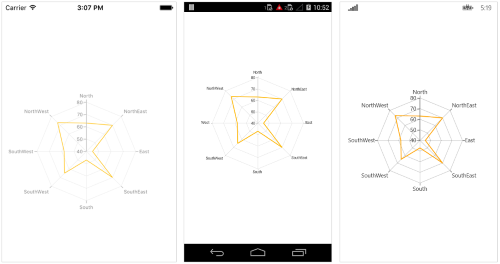### Customize the appearance

You can use the following properties to customize the appearance.

``````<chart:RadarSeries ItemsSource="{Binding RadarData}" Color="Aqua" StrokeColor="Blue"
StrokeWidth="3" XBindingPath="Name" YBindingPath="Value"  />``````
``````RadarSeries radar = new RadarSeries ();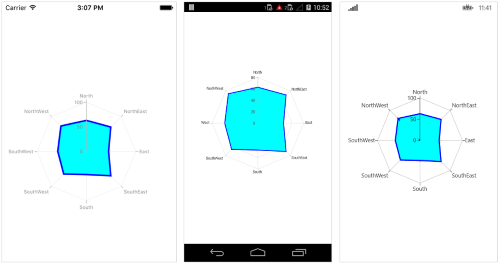### Closed

`Closed` property is used to determine, whether to connect the first and last data point of the series. By default, the property is set to `true`.

``````<chart:RadarSeries ItemsSource="{Binding RadarData}" IsClosed="false"
XBindingPath="Name" YBindingPath="Value"  />``````
``````RadarSeries radar = new RadarSeries ();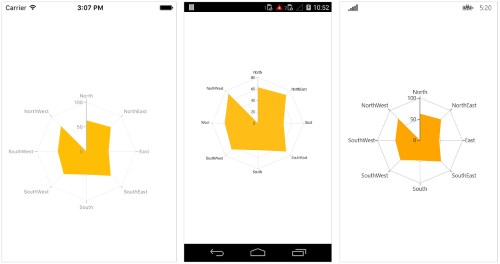### Radar start angle for primary axis

The start position of the radar series can be set by using `PolarAngle` property of axis. Default value of `PolarAngle` property is `Rotate270`. `PolarAngle` property can be set for primary axis, secondary axis, or both axes

``````<chart:SfChart.PrimaryAxis>

<chart:CategoryAxis PolarAngle = “Rotate0”/>

</chart:SfChart.PrimaryAxis >

<chart:SfChart.SecondaryAxis>

<chart:NumericalAxis/>

</chart:SfChart.SecondaryAxis >``````
``````chart.PrimaryAxis  = new CategoryAxis(){ PolarAngle = ChartPolarAngle.Rotate0 };

chart.SecondaryAxis =  new NumericalAxis();``````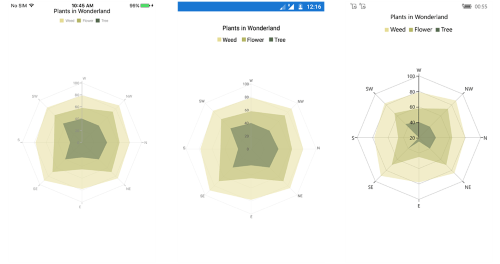### Radar start angle for secondary axis

``````<chart:SfChart.PrimaryAxis>

<chart:CategoryAxis/>

</chart:SfChart.PrimaryAxis>

<chart:SfChart.SecondaryAxis>

<chart:NumericalAxis  PolarAngle="Rotate0"/>

</chart:SfChart.SecondaryAxis>``````
``````chart.PrimaryAxis  = new CategoryAxis();

chart.SecondaryAxis =  new NumericalAxis() { PolarAngle = ChartPolarAngle.Rotate0 };``````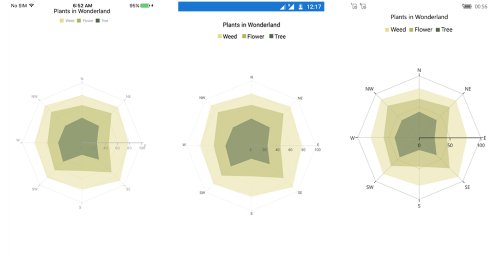### Radar start angle for both axis

``````<chart:SfChart.PrimaryAxis>

<chart:CategoryAxis PolarAngle = “Rotate0” />

</chart:SfChart.PrimaryAxis>

<chart:SfChart.SecondaryAxis>

<chart:NumericalAxis  PolarAngle = “Rotate0” />

</chart:SfChart.SecondaryAxis >``````
``````chart.PrimaryAxis  = new CategoryAxis(){ PolarAngle = ChartPolarAngle.Rotate0 };

chart.SecondaryAxis =  new NumericalAxis() { PolarAngle = ChartPolarAngle.Rotate0 };``````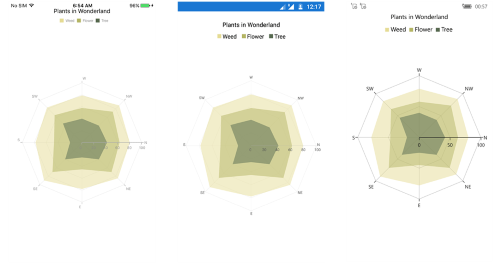NOTE

You can also explore our Xamarin Radar Chart example to know how to represent time-dependent data, showing trends in data at equal intervals.

## Polar Chart

To render a `Xamarin Polar chart`, create an instance of `PolarSeries` and add to the `Series` collection property of `SfChart`.

### Draw type

`DrawType` property is used to specify the polar series rendering type. Following are the two options you can set to this property,

``````<chart:PolarSeries ItemsSource="{Binding PolarData}" DrawType="Line"
XBindingPath="Name" YBindingPath="Value"  />``````
``````PolarSeries polar = new PolarSeries ();
polar.ItemsSource = viewModel.PolarData;
polar.XBindingPath = "Name";
polar.YBindingPath = "Value";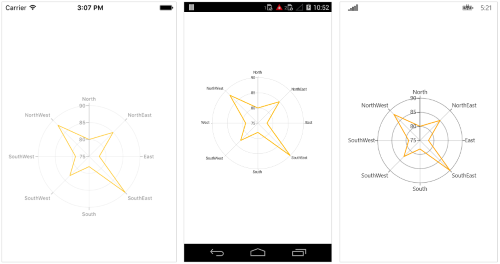### Customize the appearance

You can use the following properties to customize the appearance.

``````<chart:PolarSeries ItemsSource="{Binding PolarData}" StrokeColor="Blue" Color="Aqua"
StrokeWidth="3" XBindingPath="Name" YBindingPath="Value"  />``````
``````PolarSeries polar = new PolarSeries ();
polar.ItemsSource = viewModel.PolarData;
polar.XBindingPath = "Name";
polar.YBindingPath = "Value";
polar.Color = Color.Aqua;
polar.StrokeColor = Color.Blue;
polar.StrokeWidth = 3;``````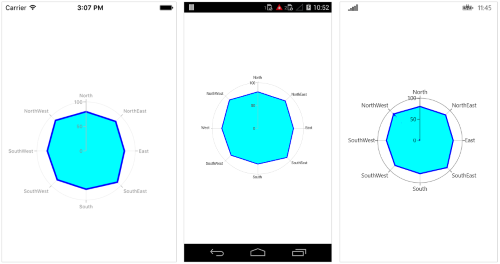### Closed

`Closed` property is used to determine, whether to connect the first and last data point of the series. By default, the property is set to `true`.

``````<chart:PolarSeries ItemsSource="{Binding PolarData}" IsClosed="false"
XBindingPath="Name" YBindingPath="Value"  />``````
``````PolarSeries polar = new PolarSeries ();
polar.ItemsSource = viewModel.PolarData;
polar.XBindingPath = "Name";
polar.YBindingPath = "Value";
polar.IsClosed = false;``````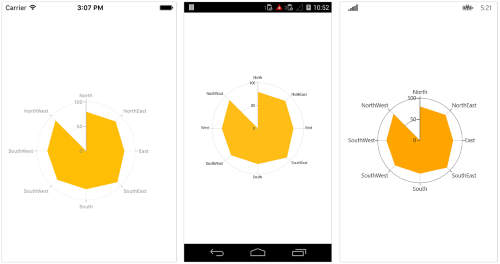### Polar start angle for primary axis

The start position of the polar series can be set by using `PolarAngle` property of axis. Default value of `PolarAngle` property is `Rotate270`. `PolarAngle` property can be set for primary axis, secondary axis, or both axes.

``````<chart:SfChart.PrimaryAxis>

<chart:CategoryAxis PolarAngle = “Rotate0”/>

</chart:SfChart.PrimaryAxis >

<chart:SfChart.SecondaryAxis>

<chart:NumericalAxis/>

</chart:SfChart.SecondaryAxis >``````
``````chart.PrimaryAxis  = new CategoryAxis(){ PolarAngle = ChartPolarAngle.Rotate0 };

chart.SecondaryAxis =  new NumericalAxis();``````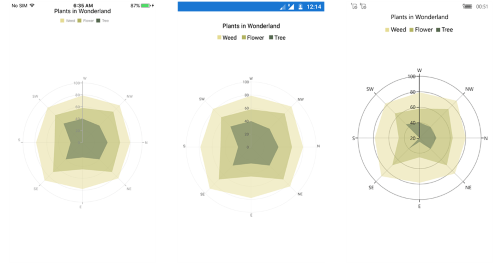### Polar start angle for secondary axis

``````<chart:SfChart.PrimaryAxis>

<chart:CategoryAxis/>

</chart:SfChart.PrimaryAxis>

<chart:SfChart.SecondaryAxis>

<chart:NumericalAxis PolarAngle="Rotate0"/>

</chart:SfChart.SecondaryAxis>``````
``````chart.PrimaryAxis  = new CategoryAxis();

chart.SecondaryAxis =  new NumericalAxis() { PolarAngle = ChartPolarAngle.Rotate0 };``````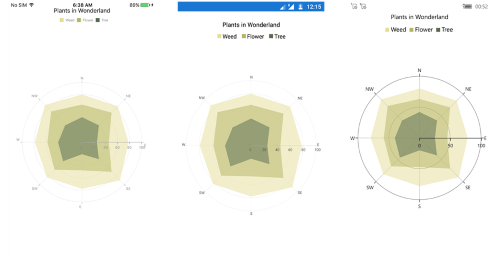### Polar start angle for both axis

``````<chart:SfChart.PrimaryAxis>

<chart:CategoryAxis PolarAngle = “Rotate0” />

</chart:SfChart.PrimaryAxis >

<chart:SfChart.SecondaryAxis>

<chart:NumericalAxis  PolarAngle = “Rotate0” />

</chart:SfChart.SecondaryAxis >``````
``````chart.PrimaryAxis  = new CategoryAxis(){ PolarAngle = ChartPolarAngle.Rotate0 };

chart.SecondaryAxis =  new NumericalAxis() { PolarAngle = ChartPolarAngle.Rotate0 };``````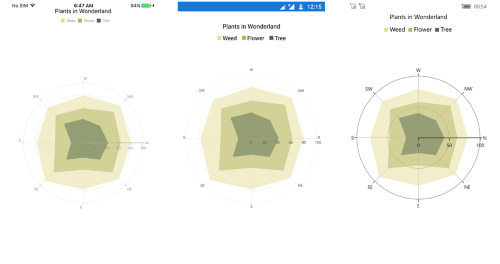NOTE

You can also explore our Xamarin Polar Chart example to know how to render and configure the polar line type charts.

## Pie Chart

To render a `Xamarin Pie chart`, create an instance of `PieSeries` and add to the `Series` collection property of `SfChart`. You can use the following properties to customize the pie segment appearance.

NOTE

ConnectorLinePosition provides better alignment to the straight connector lines with outside extended label position for the minimum number of data points.

``````<chart:SfChart>
...

<chart:PieSeries ItemsSource ="{Binding Data}" XBindingPath="Expense"
YBindingPath="Value"/>

</chart:SfChart>``````
``````SfChart chart = new SfChart();
...

PieSeries pieSeries = new PieSeries()
{
ItemsSource = Data,
XBindingPath = "Expense",
YBindingPath = "Value"
};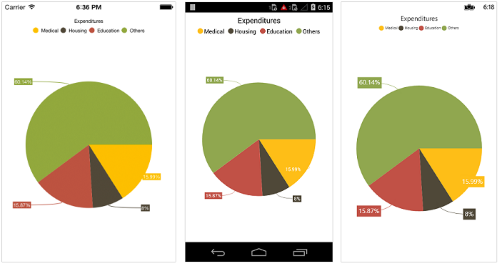NOTE

You can also explore our Xamarin Pie Chart example to know how to render and configure the pie chart.

### Changing the pie size

You can use `CircularCoefficient` property to change the diameter of the pie chart with respect to the plot area. It ranges from 0 to 1 and the default value is `0.8`.

``````<chart:PieSeries CircularCoefficient="0.5"
ItemsSource ="{Binding Data}"
XBindingPath="Expense"
YBindingPath="Value"/>``````
``````PieSeries pieSeries = new PieSeries()
{
ItemsSource = Data,
XBindingPath = "Expense",
YBindingPath = "Value",
CircularCoefficient = 0.5
};``````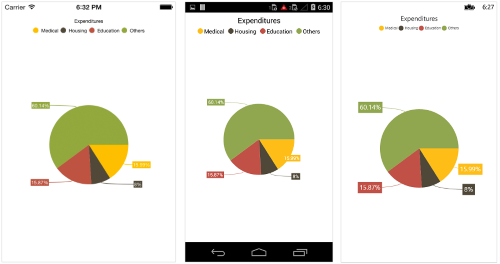### Exploding a pie segment

You can explode a pie segment using `ExplodeIndex` property and specify the explode radius using `ExplodeRadius` property of `PieSeries`.

``````<chart:PieSeries ExplodeIndex="1"
ItemsSource ="{Binding Data}"
XBindingPath="Expense"
YBindingPath="Value"/>``````
``````PieSeries pieSeries = new PieSeries()
{
ItemsSource = Data,
XBindingPath = "Expense",
YBindingPath = "Value",
ExplodeIndex = 1
};``````

Also, the segments can be exploded by touch using `ExplodeOnTouch` property of `PieSeries`. Default value of this property is false.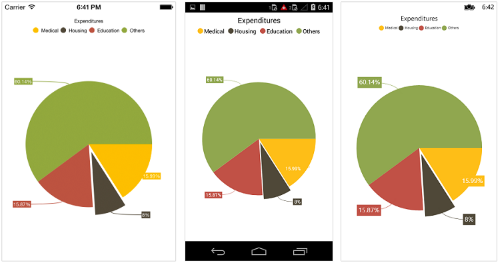### Exploding all the segments

Using `ExplodeAll` property of `PieSeries`, you can explode all the pie segments.

``````<chart:PieSeries ExplodeAll="True" ItemsSource ="{Binding Data}" XBindingPath="Expense"
YBindingPath="Value"/>``````
``````PieSeries pieSeries = new PieSeries()
{
ItemsSource = Data,
XBindingPath = "Expense",
YBindingPath = "Value",
ExplodeAll = true
};``````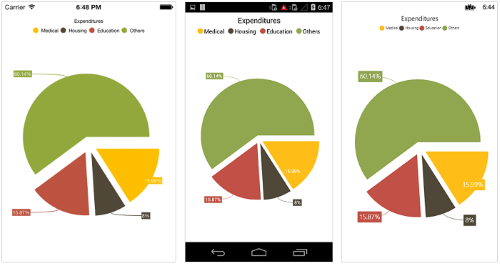### Sector of Pie

SfChart allows you to render all the data points/segments in semi-pie, quarter-pie or in any sector using `StartAngle` and `EndAngle` properties.

``````<chart:PieSeries StartAngle="180"
EndAngle="360"
ItemsSource ="{Binding Data}"
XBindingPath="Expense"
YBindingPath="Value"/>``````
``````PieSeries pieSeries = new PieSeries()
{
ItemsSource = Data,
XBindingPath = "Expense",
YBindingPath = "Value",
StartAngle = 180,
EndAngle = 360
};``````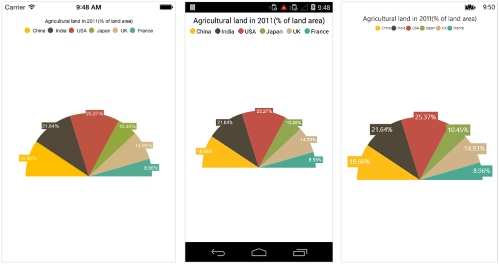### Group small data points into “others”

The small segments in the pie chart can be grouped into “others” category using the `GroupTo` and `GroupMode` properties of PieSeries. The `GroupMode` property is used to specify the grouping type based on slice `Angle`, actual data point value, or `Percentage`, and the `GroupTo` property is used to set the limit to group data points into a single slice. The grouped segment is labeled as “Others” in legend and toggled as any other segment. The default value of the `GroupTo` property and `GroupMode` property is Value.

## Doughnut Chart

To render a `Xamarin Doughnut Chart`, create an instance of `DoughnutSeries` and add to the `Series` collection property of `SfChart`. You can use the following properties to customize the doughnut segment appearance.

NOTE

ConnectorLinePosition provides better alignment to the straight connector lines with outside extended label position for the minimum number of data points.

``````<chart:SfChart>
...

<chart:DoughnutSeries ItemsSource ="{Binding Data}"
XBindingPath="Expense"
YBindingPath="Value"/>

</chart:SfChart>``````
``````SfChart chart = new SfChart();
...

DoughnutSeries doughnutSeries = new DoughnutSeries()
{
ItemsSource = Data,
XBindingPath = "Expense",
YBindingPath = "Value"
};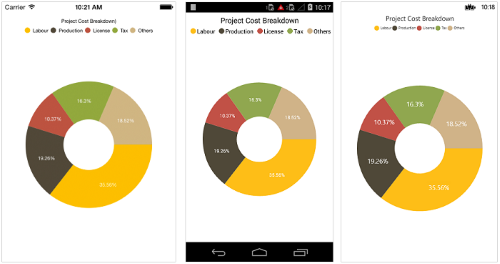NOTE

You can also explore our Xamarin Doughnut Chart example to knows how to render and configure the donut charts.

### Stacked doughnut

Doughnut segments can be separated as individual circles using the `IsStackedDoughnut` property. The following properties are used to customize the stacked doughnut chart:

``````<chart:SfChart>
...

<chart:DoughnutSeries ItemsSource ="{Binding Data}"
XBindingPath="Expense"
YBindingPath="Value"
IsStackedDoughnut="true"
CapStyle="BothCurve"
Spacing=”0.4”
MaximumValue=“100” />

</chart:SfChart>``````
``````SfChart chart = new SfChart();
...

DoughnutSeries doughnutSeries = new DoughnutSeries()
{
ItemsSource = Data,
XBindingPath = "Expense",
YBindingPath = "Value",
IsStackedDoughnut = true,
CapStyle = DoughnutCapStyle.BothCurve,
Spacing = 0.4,
MaximumValue = 100
};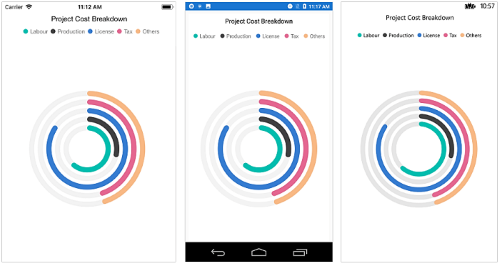You can change the doughnut chart inner radius using `DoughnutCoefficient` with respect to the plot area. It ranges from 0 to 1 and the default value is `0.4`.

``````<chart:DoughnutSeries DoughnutCoefficient="0.6"
ItemsSource ="{Binding Data}"
XBindingPath="Expense"
YBindingPath="Value" />``````
``````DoughnutSeries doughnutSeries = new DoughnutSeries()
{
ItemsSource = Data,
XBindingPath = "Expense",
YBindingPath = "Value",
DoughnutCoefficient = 0.6
};``````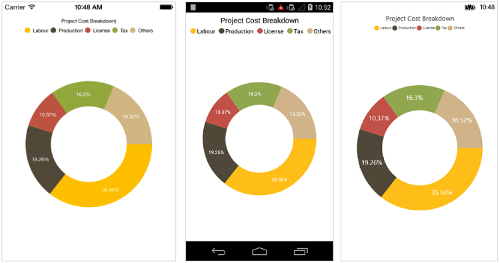### Changing the doughnut size

You can use the `CircularCoefficient` property to change the diameter of the doughnut chart with respect to the plot area. It ranges from 0 to 1 and the default value is 0.8.

``````<chart:DoughnutSeries CircularCoefficient="0.5"
ItemsSource ="{Binding Data}"
XBindingPath="Expense"
YBindingPath="Value"/>``````
``````DoughnutSeries doughnutSeries = new DoughnutSeries()
{
ItemsSource = Data,
XBindingPath = "Expense",
YBindingPath = "Value",
CircularCoefficient = 0.5
};``````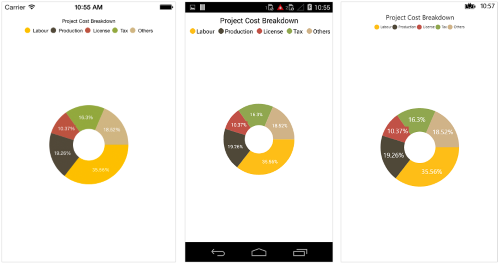### Exploding a doughnut segment

Exploding a specific doughnut segment, you have to set the index to be exploded using `ExplodeIndex` property of the series.

``````<chart:DoughnutSeries ExplodeIndex="1" ItemsSource ="{Binding Data}" XBindingPath="Expense"
YBindingPath="Value" />``````
``````DoughnutSeries doughnutSeries = new DoughnutSeries()
{
ItemsSource = Data,
XBindingPath = "Expense",
YBindingPath = "Value",
ExplodeIndex = 1
};``````

Also, the segments can be exploded by touch using `ExplodeOnTouch` property of `DoughnutSeries`. Default value of this property is false.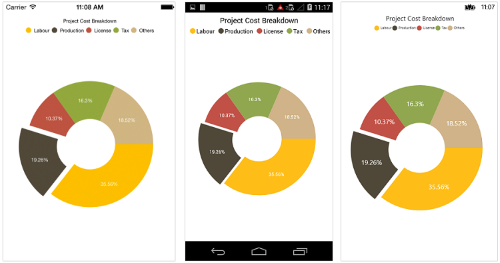### Exploding all the segments

To explode all the segments, you have to enable `ExplodeAll` property of the series.

``````<chart:DoughnutSeries ExplodeAll="True" ItemsSource ="{Binding Data}" XBindingPath="Expense"
YBindingPath="Value" />``````
``````DoughnutSeries doughnutSeries = new DoughnutSeries()
{
ItemsSource = Data,
XBindingPath = "Expense",
YBindingPath = "Value",
ExplodeAll = true
};``````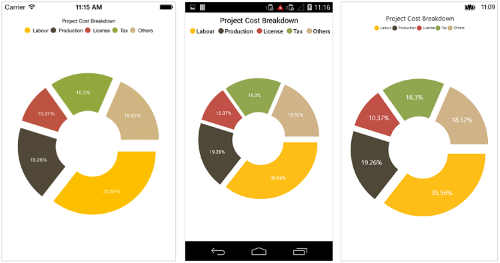### Sector of Doughnut

`SfChart` allows you to render all the data points/segments in semi-doughnut, quarter- doughnut or in any sector using `StartAngle` and `EndAngle` properties.

``````<chart:DoughnutSeries StartAngle="180" EndAngle="360" ItemsSource ="{Binding Data}"
XBindingPath="Expense" YBindingPath="Value" />``````
``````DoughnutSeries doughnutSeries = new DoughnutSeries()
{
ItemsSource = Data,
XBindingPath = "Expense",
YBindingPath = "Value",
StartAngle = 180,
EndAngle = 360
};``````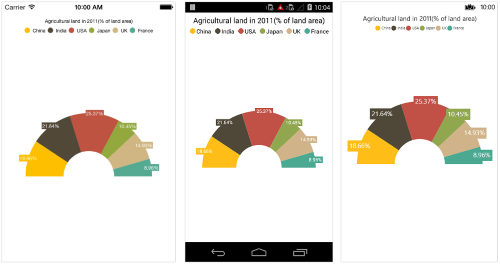### Group small data points into “others”

The small segments in the doughnut chart can be grouped into “others” category using the `GroupTo` and `GroupMode` properties of DoughnutSeries. The `GroupMode` property is used to specify the grouping type based on slice angle, actual data point value, or percentage, and the `GroupTo` property is used to set the limit to group data points into a single slice. The grouped segment is labeled as “Others” in legend and toggled as any other segment. The default value of the `GroupTo` property and `GroupMode` property is Value.

### Add view to the center of doughnut chart

Any view can be added to the center of doughnut chart using the `CenterView` property of `DoughnutSeries`. The binding context of the `CenterView` will be the respective `DoughnutSeries`.

``````<chart:DoughnutSeries>
...

<chart:DoughnutSeries.CenterView>
<StackLayout HorizontalOptions = "FillAndExpand"
VerticalOptions = "FillAndExpand">
...
</StackLayout>
</chart:DoughnutSeries.CenterView>
...
</chart:DoughnutSeries>``````
``````DoughnutSeries doughnutSeries = new DoughnutSeries()
{
...
}
doughnutSeries.CenterView = new Label() { Text = "CenterView" };``````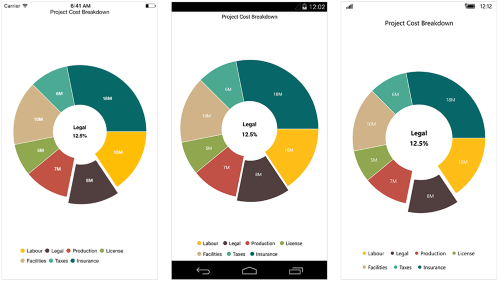The `InnerRadius` property of `DoughnutSeries` is used to get only the inner radius. Using this `InnerRadius` value, you can provide `CentreView` for series to avoid the view from being cropped outside the series.

## Pyramid Chart

To render a `Xamarin Pyramid chart`, create an instance of `PyramidSeries` and add to the `Series` collection property of `SfChart`. You can use the following properties to customize the pyramid segment appearance.

``````<chart:SfChart>
...

<chart:PyramidSeries ItemsSource ="{Binding Data}" XBindingPath="Country"
YBindingPath="Value"/>

</chart:SfChart>``````
``````SfChart chart = new SfChart();
...

PyramidSeries pyramidSeries = new PyramidSeries()
{
ItemsSource = Data,
XBindingPath = "Country",
YBindingPath = "Value"
};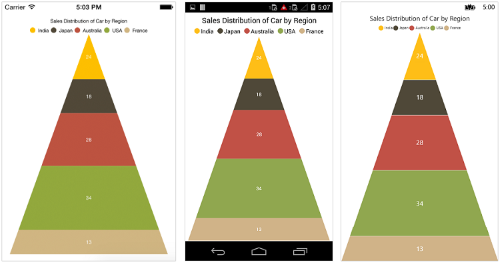NOTE

. You can also explore our Xamarin Pyramid Chart example to know how to render a pyramid series.

### Pyramid Mode

You can render the pyramid series as linear or surface mode. In linear mode, height of the pyramid segment is based on the Y value and in surface mode, area of the pyramid segment is based on the Y values. The default value of `PyramidMode` property is `Linear`.

``````<chart:PyramidSeries ItemsSource ="{Binding Data}" PyramidMode="Surface" XBindingPath="Country"
YBindingPath="Value"/>``````
``````PyramidSeries pyramidSeries = new PyramidSeries ()
{
ItemsSource = Data,
XBindingPath = "Country",
YBindingPath = "Value",
PyramidMode = ChartPyramidMode.Surface
};``````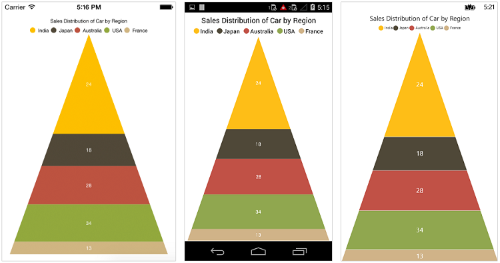### Gap between the segments

You can control the gap between the two segments using `GapRatio` property. Its ranges from 0 to 1.

``````<chart:PyramidSeries ItemsSource ="{Binding Data}" GapRatio="0.1"
XBindingPath="Country"
YBindingPath="Value"/>``````
``````PyramidSeries pyramidSeries = new PyramidSeries()
{
ItemsSource = Data,
XBindingPath = "Country",
YBindingPath = "Value",
GapRatio = 0.1
};``````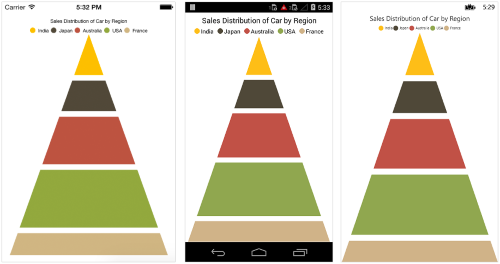### Exploding a pyramid segment

You can explode a pyramid segment using `ExplodeIndex` property, and `ExplodeOffset` property is used to specify the exploded segment’s distance.

``````<chart:PyramidSeries ItemsSource ="{Binding Data}" ExplodeIndex="2"
XBindingPath="Country"
YBindingPath="Value" />``````
``````PyramidSeries pyramidSeries = new PyramidSeries()
{
ItemsSource = Data,
XBindingPath = "Country",
YBindingPath = "Value",
ExplodeIndex = 2
};``````
Also, the segments can be exploded by touch using [`ExplodeOnTouch`](https://help.syncfusion.com/cr/xamarin/Syncfusion.SfChart.XForms.AccumulationSeries.html#Syncfusion_SfChart_XForms_AccumulationSeries_ExplodeOnTouch) property of [`PyramidSeries`](https://help.syncfusion.com/cr/xamarin/Syncfusion.SfChart.XForms.PyramidSeries.html). Default value of this property is false.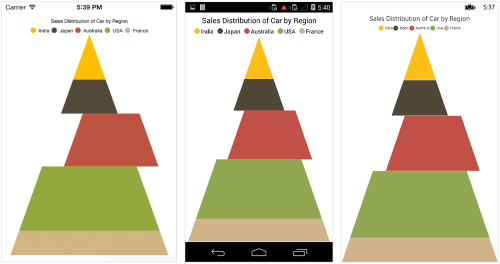## Funnel Chart

To render a `Xamarin Funnel Chart`, create an instance of `FunnelSeries` and add to the `Series` collection property of `SfChart`. You can use the following properties to customize the funnel segment appearance.

``````<chart:SfChart>
...

<chart:FunnelSeries ItemsSource ="{Binding Data}" XBindingPath="Status"
YBindingPath="Value"/>

</chart:SfChart>``````
``````SfChart chart = new SfChart();
...

FunnelSeries funnelSeries = new FunnelSeries()
{
ItemsSource = Data,
XBindingPath = "Status",
YBindingPath = "Value"
};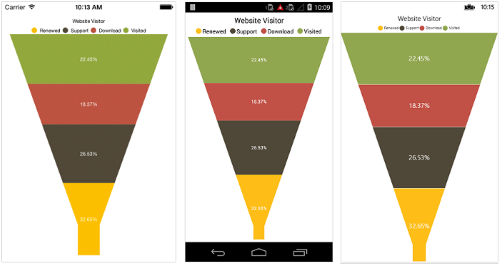NOTE

You can also explore our Xamarin Funnel Chart example to knows how to render and configure the funnel chart.

### Gap between the segments

You can control the gap between the two segments using `GapRatio` property. Its ranges from 0 to 1.

``````<chart:FunnelSeries ItemsSource ="{Binding Data}" GapRatio="0.1"
XBindingPath="Year"
YBindingPath="Value"/>``````
``````FunnelSeries funnelSeries = new FunnelSeries()
{
ItemsSource = Data,
XBindingPath = "Year",
YBindingPath = "Value",
GapRatio = 0.1
};``````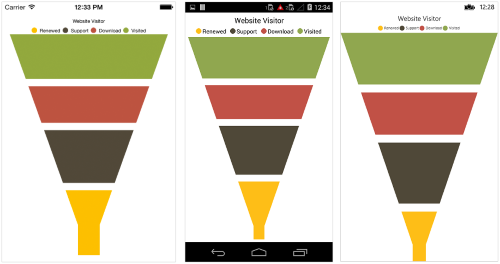### Exploding a funnel segment

You can explode a pyramid segment using `ExplodeIndex` property and `ExplodeOffset` property is used to specify the exploded segment’s distance.

``````<chart:FunnelSeries ItemsSource ="{Binding Data}" ExplodeIndex="1"
XBindingPath="Status"
YBindingPath="Value" />``````
``````FunnelSeries funnelSeries = new FunnelSeries()
{
ItemsSource = Data,
XBindingPath = "Status",
YBindingPath = "Value",
ExplodeIndex = 1
};``````

Also, the segments can be exploded by touch using `ExplodeOnTouch` property of `FunnelSeries`. Default value of this property is false.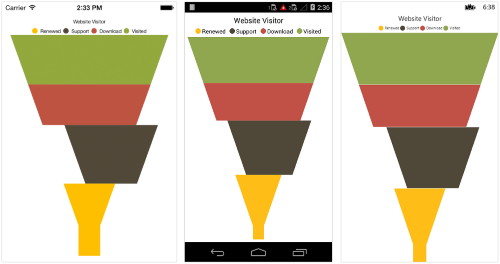### Changing the minimum width of the funnel

You can change the minimum width of the funnel neck using `MinWidth` property of `FunnelSeries`. Default value of minWidth is `40`.

``````<chart:FunnelSeries ItemsSource ="{Binding Data}" MinWidth="20"
XBindingPath="Year"
YBindingPath="Value"/>``````
``````FunnelSeries funnelSeries = new FunnelSeries()
{
ItemsSource = Data,
XBindingPath = "Year",
YBindingPath = "Value",
MinWidth = 20
};``````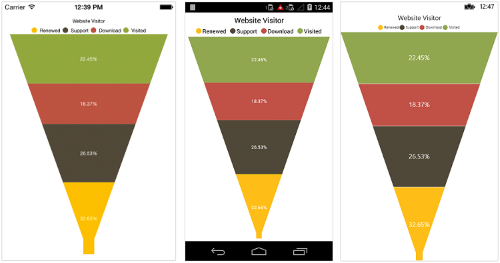## Waterfall Chart

`WaterfallSeries` clarifies the cumulative effect of a set of provided positive and negative values. The series is represented by a rectangle and a connector between the rectangles.

``````<chart:WaterfallSeries ItemsSource="{Binding RevenueDetails}"
XBindingPath="Category"
YBindingPath="Value"
AllowAutoSum="True"
SummaryBindingPath="IsSummary"
NegativeSegmentColor="#F14C72"
SummarySegmentColor="#8C8C8C"
ShowConnectorLine="True">
</chart:WaterfallSeries>``````
``````WaterfallSeries waterfallSeries = new WaterfallSeries()
{
ItemsSource = RevenueDetails,
XBindingPath = "Category",
YBindingPath = "Value",
AllowAutoSum = true,
SummaryBindingPath = "IsSummary",
NegativeSegmentColor = Color.FromHex("#F14C72"),
SummarySegmentColor = Color.FromHex("#8C8C8C"),
ShowConnectorLine = true
};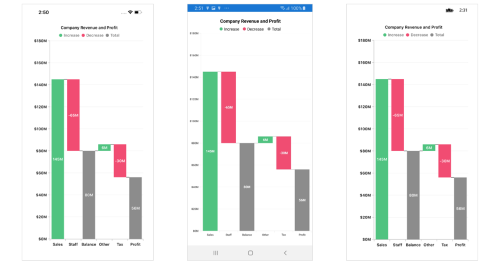## Box and Whisker Chart

BoxAndWhiskerSeries plots a combination of rectangles and lines to show the distribution of data sets. To render a box and whisker(box plot) chart, create an instance of `BoxAndWhiskerSeries` and add to the `Series` collection property of `SfChart`. You can use the following properties to customize the appearance.

• `Color` - used to change the color of the series.
• `StrokeWidth` - used to change the stroke width of the series.
• `StrokeColor` - used to change the stroke color of the series.
• `Spacing` - used to change the spacing between two segments. The default value of spacing is 0, and the value ranges from 0 to 1. Here, 1 and 0 corresponds to 100% and 0% of the available space, respectively.
• `Width` - used to change the width of the rectangle. The default value of the width is 0.8, and the value ranges from 0 to 1. Here, 1 and 0 corresponds to 100% and 0% of the available width, respectively.
``````<chart:SfChart>
...

<chart:BoxAndWhiskerSeries ItemSource ="{Binding Data"} XBindingPath="Department"
YBindingPath="Ages"
ShowMedian="True" />

</chart:SfChart>``````
``````SfChart chart = new SfChart();
...

BoxAndWhiskerSeries boxPlotSeries = new BoxAndWhiskerSeries()
{
ItemsSource = Data,
XBindingPath = "Department",
YBindingPath = "Ages",
ShowMedian = true;
};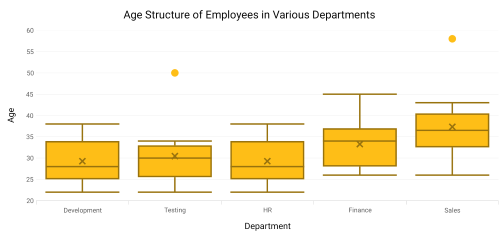### Customize the series box mode

The series box plotting mode can be changed using the `BoxPlotMode` property of `BoxAndWhiskerSeries`. The plotting mode of series can be calculated as follows:

• `Exclusive` - The quartile values are calculated using the formula (N+1) * P (N count, P percentile), and their index value starts from 1 in the list.
• `Inclusive` - The quartile values are calculated using the formula (N−1) * P (N count, P percentile), and their index value starts from 0 in the list.
• `Normal` - The quartile values are calculated by splitting the list and getting the median values.

By default, `BoxPlotMode` value is `Exclusive`.

The following code shows how to set the `BoxPlotMode` value as `Inclusive`.

``````<chart:SfChart>
...

<chart:BoxAndWhiskerSeries ItemSource ="{Binding Data"} XBindingPath="Department"
YBindingPath="Ages"
BoxPlotMode="Inclusive"
ShowMedian="True" />

</chart:SfChart>``````
``````SfChart chart = new SfChart();
...

BoxAndWhiskerSeries boxPlotSeries = new BoxAndWhiskerSeries()
{
ItemsSource = Data,
XBindingPath = "Department",
YBindingPath = "Ages",
BoxPlotMode = BoxPlotMode.Inclusive,
ShowMedian = true
};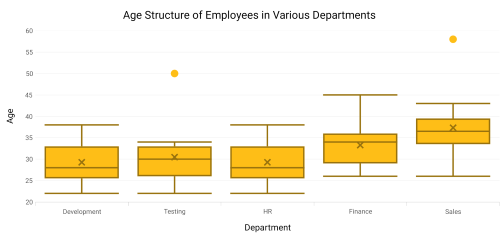### ShowMedian

The Median values of given data set is viewed by enabling the `ShowMedian` property of `BoxAndWhiskerSeries`. By default,`ShowMedian` value is false. The following code demonstrates how to enable the `ShowMedian` property.

``````<chart:SfChart>
...

<chart:BoxAndWhiskerSeries ItemSource ="{Binding Data"} XBindingPath="Department"
YBindingPath="Ages"
ShowMedian="True" />

</chart:SfChart>``````
``````SfChart chart = new SfChart();
...

BoxAndWhiskerSeries boxPlotSeries = new BoxAndWhiskerSeries()
{
ItemsSource = Data,
XBindingPath = "Department",
YBindingPath = "Ages",
ShowMedian = true
}### Outlier

The outlier is used to display the outlier point that lies either below the lower whisker or above the upper whisker line and it is an observation that is numerically distant from the rest of the data.

#### ShowOutlier

The outlier value in the box plot can be viewed by enabling the `ShowOutlier` property of `BoxAndWhiskerSeries`. By default, ShowOutlier value is true. The following code demonstrates how to disable the `ShowOutlier` property.

``````<chart:SfChart>
...

<chart:BoxAndWhiskerSeries ItemSource ="{Binding Data"} XBindingPath="Department"
YBindingPath="Ages"
ShowMedian="True"
ShowOutlier="False" />

</chart:SfChart>``````
``````SfChart chart = new SfChart();
...

BoxAndWhiskerSeries boxPlotSeries = new BoxAndWhiskerSeries()
{
ItemsSource = Data,
XBindingPath = "Department",
YBindingPath = "Ages",
ShowMedian = true,
ShowOutlier = false
}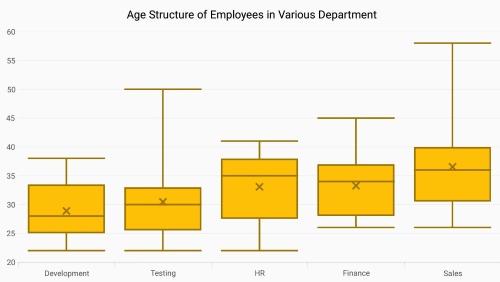### SymbolType

The `SymbolType` is used to display the outlier point with different types of symbols. The available symbols are Cross, Diamond, Ellipse, Hexagon, InvertedTriangle, Pentagon, Plus, Rectangle and Triangle. By default, `SymbolType` value is `Ellipse`.

The following code shows how to set the `SymbolType` value as `Cross`.

``````<chart:SfChart>
...

<chart:BoxAndWhiskerSeries ItemSource ="{Binding Data"} XBindingPath="Department"
YBindingPath="Ages"
ShowMedian="True"
SymbolType="Cross" />

</chart:SfChart>``````
``````SfChart chart = new SfChart();
...

BoxAndWhiskerSeries boxPlotSeries = new BoxAndWhiskerSeries()
{
ItemsSource = Data,
XBindingPath = "Department",
YBindingPath = "Ages",
ShowMedian = true,
SymbolType = ChartSymbolType.Cross
}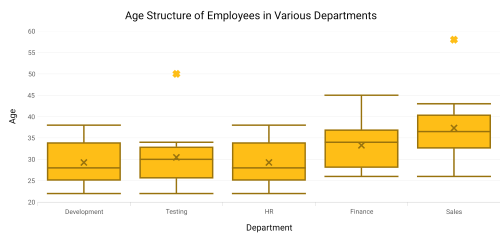## Error Bar Chart

ErrorBarSeries is graphical representations of the variations of data and used on graphs to indicate the errors or uncertainty in a reported measurement. To render a error bar chart, create an instance of `ErrorBarSeries` and add to the `Series` collection property of `SfChart`.

``````<chart:SfChart>
...

<chart:ScatterSeries ScatterHeight="20"
ScatterWidth="20"
ShapeType="Ellipse"
ItemsSource ="{Binding CarDistributionDetails}"
XBindingPath="Country"
YBindingPath="Value">
<chart:ScatterSeries.ColorModel>
<chart:ChartColorModel Palette="Natural"/>
</chart:ScatterSeries.ColorModel>
</chart:ScatterSeries>

<chart:ErrorBarSeries ItemsSource = "{Binding CarDistributionDetails}"
XBindingPath = "Country"
YBindingPath = "Value"
Type = ErrorBarType.Fixed
Mode = ErrorBarMode.Both
HorizontalErrorValue = 0.5
VerticalErrorValue = 4/>

</chart:SfChart>``````
``````SfChart chart = new SfChart();
...

ScatterSeries scatterSeries = new ScatterSeries ()
{
ItemsSource = Data,
ScatterHeight = 20,
ScatterWidth = 20,
ShapeType = ChartScatterShapeType.Ellipse,
XBindingPath = "Year",
YBindingPath = "Value"
};
scatterSeries.ColorModel.Palette = ChartColorPalette.Natural;

ErrorBarSeries errorBarSeries = new ErrorBarSeries()
{
ItemsSource = CarDistributionDetails,
XBindingPath = "Country",
YBindingPath = "Value",
Type = ErrorBarType.Fixed,
Mode = ErrorBarMode.Both,
HorizontalErrorValue = 0.5,
VerticalErrorValue = 4
};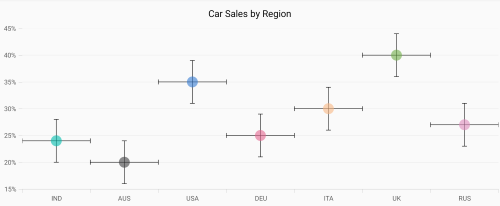### Type

The `Type` property is used to define the error bar type value in Fixed, Custom, Percentage, StandardDeviation, and StandardErrors. The default value of this property is `Fixed`. For all types, You have to set the values for `HorizontalErrorValue` and `VerticalErrorValue` except `Custom`.

#### Fixed

``````<chart:SfChart>
...

<chart:ErrorBarSeries ItemsSource = "{Binding CarDistributionDetails}"
XBindingPath = "Country"
YBindingPath = "Value"
Type = ErrorBarType.Fixed
Mode = ErrorBarMode.Both
HorizontalErrorValue = 0.5
VerticalErrorValue = 4/>

</chart:SfChart>``````
``````ErrorBarSeries errorBarSeries = new ErrorBarSeries()
{
ItemsSource = CarDistributionDetails,
XBindingPath = "Country",
YBindingPath = "Value",
Type = ErrorBarType.Fixed,
Mode = ErrorBarMode.Both,
HorizontalErrorValue = 0.5,
VerticalErrorValue = 4
};``````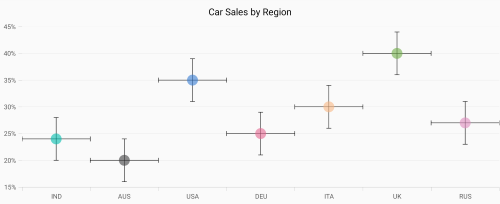#### Percentage

``````<chart:SfChart>
...

<chart:ErrorBarSeries ItemsSource = {Binding  CarDistributionDetails}"
XBindingPath = "Country"
YBindingPath = "Value"
Type = ErrorBarType.Percentage
Mode = ErrorBarMode.Both
HorizontalErrorValue = 1
VerticalErrorValue = 8/>

</chart:SfChart>``````
``````ErrorBarSeries errorBarSeries = new ErrorBarSeries()
{
ItemsSource = CarDistributionDetails,
XBindingPath = "Country",
YBindingPath = "Value",
Type = ErrorBarType.Percentage,
Mode = ErrorBarMode.Both,
HorizontalErrorValue = 1,
VerticalErrorValue = 8
};``````#### Standard Deviation

``````<chart:SfChart>
...

<chart:ErrorBarSeries ItemsSource = "{Binding  CarDistributionDetails}"
XBindingPath = "Country"
YBindingPath = "Value"
Type = ErrorBarType.StandardDeviation
Mode = ErrorBarMode.Both
HorizontalErrorValue = 1
VerticalErrorValue = 4/>

</chart:SfChart>``````
``````ErrorBarSeries errorBarSeries = new ErrorBarSeries()
{
ItemsSource = CarDistributionDetails,
XBindingPath = "Country",
YBindingPath = "Value",
Type = ErrorBarType.StandardDeviation,
Mode = ErrorBarMode.Both,
HorizontalErrorValue = 1,
VerticalErrorValue = 4
};``````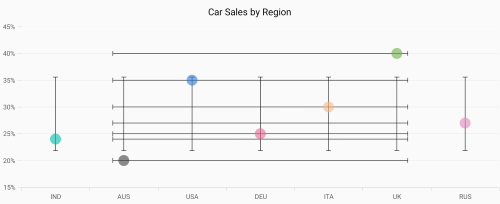#### Standard Errors

``````<chart:SfChart>
...

<chart:ErrorBarSeries ItemsSource = "{Binding CarDistributionDetails}"
XBindingPath = "Country"
YBindingPath = "Value"
Type = ErrorBarType.StandardErrors
Mode = ErrorBarMode.Both
HorizontalErrorValue = 1
VerticalErrorValue = 4/>

</chart:SfChart>``````
``````ErrorBarSeries errorBarSeries = new ErrorBarSeries()
{
ItemsSource = CarDistributionDetails,
XBindingPath = "Country",
YBindingPath = "Value",
Type = ErrorBarType.StandardErrors,
Mode = ErrorBarMode.Both,
HorizontalErrorValue = 1,
VerticalErrorValue = 4
};``````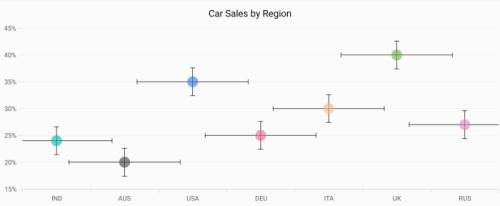#### Custom

If `Type` is `Custom`, you have to set values for `HorizontalErrorPath` and `VerticalErrorPath` as shown in the following code snippet.

``````<chart:SfChart>
...

<chart:ErrorBarSeries ItemsSource = "{Binding CarDistributionDetails}"
XBindingPath = "Country"
YBindingPath = "Value"
Type=ErrorBarType.Custom
Mode = ErrorBarMode.Both
HorizontalErrorPath = "HorizontalErrorValues"
VerticalErrorPath = "VerticalErrorValues"/>

</chart:SfChart>``````
``````ErrorBarSeries errorBarSeries = new ErrorBarSeries()
{
ItemsSource = CarDistributionDetails,
XBindingPath = "Country",
YBindingPath = "Value",
Type = ErrorBarType.Custom,
Mode = ErrorBarMode.Both,
HorizontalErrorPath = "HorizontalErrorValues",
VerticalErrorValue = "VerticalErrorValues"
};``````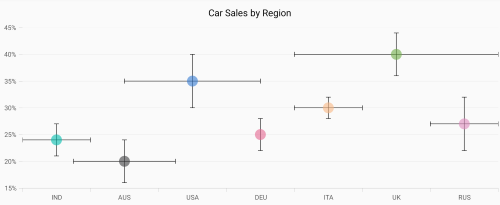### Mode

The error value shown on the chart is based on the `Mode` property. It have the values of `Both`, `Horizontal`, and `Vertical`. The default value of this property is `Both`.

#### Both

To display horizontal and vertical error values, you can set the `Mode` as `Both` as shown in the following code.

``````<chart:SfChart>
...

<chart:ErrorBarSeries ItemsSource="{Binding CarDistributionDetails}"
XBindingPath = "Country"
YBindingPath = "Value"
Type = ErrorBarType.Fixed
Mode = ErrorBarMode.Both
HorizontalErrorValue = 0.5
VerticalErrorValue = 4/>

</chart:SfChart>``````
``````ErrorBarSeries errorBarSeries = new ErrorBarSeries()
{
ItemsSource = CarDistributionDetails,
XBindingPath = "Country",
YBindingPath = "Value",
Type = ErrorBarType.Fixed,
Mode = ErrorBarMode.Horizontal,
HorizontalErrorValue = 0.5,
VerticalErrorValue = 4
};``````#### Horizontal

To display horizontal error value only, you can set the `Mode` as `Horizontal` as shown in the following code.

``````<chart:SfChart>
...

<chart:ErrorBarSeries ItemsSource="{Binding CarDistributionDetails}"
XBindingPath = "Country"
YBindingPath = "Value"
Type = ErrorBarType.Fixed
Mode = ErrorBarMode.Horizontal
HorizontalErrorValue = 0.5
VerticalErrorValue = 4/>

</chart:SfChart>``````
``````ErrorBarSeries errorBarSeries = new ErrorBarSeries()
{
ItemsSource = CarDistributionDetails,
XBindingPath = "Country",
YBindingPath = "Value",
Type = ErrorBarType.Fixed,
Mode = ErrorBarMode.Horizontal,
HorizontalErrorValue = 0.5,
VerticalErrorValue = 4
};``````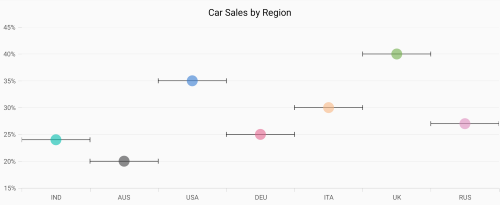#### Vertical

To display vertical error value only, you can set the `Mode` as `Vertical` as shown in the following code.

``````<chart:SfChart>
...

<chart:ErrorBarSeries ItemsSource="{Binding CarDistributionDetails}"
XBindingPath = "Country"
YBindingPath = "Value"
Type = ErrorBarType.Fixed
Mode = ErrorBarMode.Vertical
HorizontalErrorValue = 0.5
VerticalErrorValue = 4/>

</chart:SfChart>``````
``````ErrorBarSeries errorBarSeries = new ErrorBarSeries()
{
ItemsSource = CarDistributionDetails,
XBindingPath = "Country",
YBindingPath = "Value",
Type = ErrorBarType.Fixed,
Mode = ErrorBarMode.Vertical,
HorizontalErrorValue = 0.5,
VerticalErrorValue = 4
};``````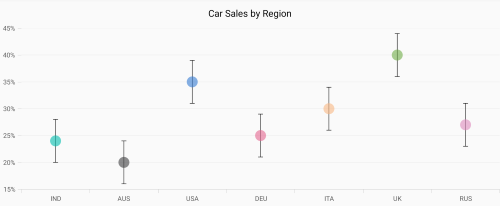### Direction

The `HorizontalDirection` and `VerticalDirection` properties are used to set the direction of error bar lines. The default value is `Both`.

``````<chart:SfChart>
...

<chart:ErrorBarSeries ItemsSource = "{Binding CarDistributionDetails}"
XBindingPath = "Country"
YBindingPath = "Value"
HorizontalDirection = ErrorBarDirection.Plus
VerticalDirection = ErrorBarDirection.Minus
HorizontalErrorValue = 0.5
VerticalErrorValue = 4/>

</chart:SfChart>``````
``````ErrorBarSeries errorBarSeries = new ErrorBarSeries()
{
ItemsSource = CarDistributionDetails,
XBindingPath = "Country",
YBindingPath = "Value",
HorizontalDirection = ErrorBarDirection.Plus,
VerticalDirection = ErrorBarDirection.Minus,
HorizontalErrorValue = 0.5,
VerticalErrorValue = 4
};``````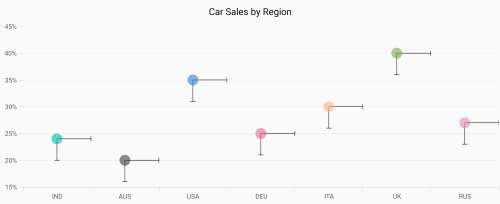### Customization

You can customize the `ErrorBarSeries` with the following style properties.

``````<chart:SfChart>
...

<chart:ErrorBarSeries.HorizontalLineStyle>
<chart:ErrorBarLineStyle
StrokeColor= "Cyan"
StrokeWidth = "4">
</chart:ErrorBarSeries.HorizontalLineStyle>

<chart:ErrorBarSeries.VerticalLineStyle>
<chart:ErrorBarLineStyle
StrokeColor = "Cyan"
StrokeWidth = "4">
</chart:ErrorBarSeries.VerticalLineStyle>

<chart:ErrorBarSeries.HorizontalCapLineStyle>
<chart:ErrorBarCapLineStyle
StrokeColor= "Green"
StrokeWidth= "4"
IsVisible = "true">
</chart:ErrorBarSeries.HorizontalCapLineStyle>

<chart:ErrorBarSeries.VerticalCapLineStyle>
<chart:ErrorBarCapLineStyle
StrokeColor = "Green"
StrokeWidth = "4"
IsVisible = "true">
</chart:ErrorBarSeries.VerticalCapLineStyle>

</chart:SfChart>``````
``````errorBarSeries.HorizontalLineStyle = new ErrorBarLineStyle();
errorBarSeries.HorizontalLineStyle.StrokeColor = Color.Cyan;
errorBarSeries.HorizontalLineStyle.StrokeWidth = 4;

errorBarSeries.VerticalLineStyle = new ErrorBarLineStyle();
errorBarSeries.VerticalLineStyle.StrokeColor = Color.Cyan;
errorBarSeries.VerticalLineStyle.StrokeWidth = 4;

errorBarSeries.HorizontalCapLineStyle = new ErrorBarCapLineStyle();
errorBarSeries.HorizontalCapLineStyle.StrokeColor = Color.Green;
errorBarSeries.HorizontalCapLineStyle.StrokeWidth = 4;
errorBarSeries.HorizontalCapLineStyle.IsVisible = true;

errorBarSeries.VerticalCapLineStyle = new ErrorBarCapLineStyle();
errorBarSeries.VerticalCapLineStyle.StrokeColor = Color.Green;
errorBarSeries.VerticalCapLineStyle.StrokeWidth = 4;
errorBarSeries.VerticalCapLineStyle.IsVisible = true;``````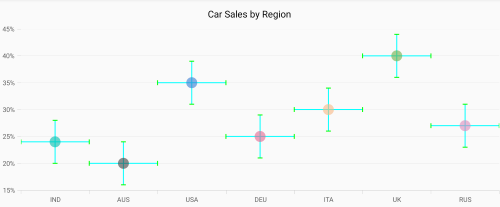NOTE

You can refer to our Xamarin Charts feature tour page for its groundbreaking feature representations. You can also explore our Xamarin.Forms Charts example to knows various chart types and how to easily configured with built-in support for creating stunning visual effects.